Last updated: 2020-08-23

Checks: 7 0

Knit directory: r4ds_book/

This reproducible R Markdown analysis was created with workflowr (version 1.6.2). The Checks tab describes the reproducibility checks that were applied when the results were created. The Past versions tab lists the development history.

Great! Since the R Markdown file has been committed to the Git repository, you know the exact version of the code that produced these results.

Great job! The global environment was empty. Objects defined in the global environment can affect the analysis in your R Markdown file in unknown ways. For reproduciblity it’s best to always run the code in an empty environment.

The command set.seed(20200814) was run prior to running the code in the R Markdown file. Setting a seed ensures that any results that rely on randomness, e.g. subsampling or permutations, are reproducible.

Great job! Recording the operating system, R version, and package versions is critical for reproducibility.

Nice! There were no cached chunks for this analysis, so you can be confident that you successfully produced the results during this run.

Great job! Using relative paths to the files within your workflowr project makes it easier to run your code on other machines.

Great! You are using Git for version control. Tracking code development and connecting the code version to the results is critical for reproducibility.

The results in this page were generated with repository version b96b2ff. See the Past versions tab to see a history of the changes made to the R Markdown and HTML files.

Note that you need to be careful to ensure that all relevant files for the analysis have been committed to Git prior to generating the results (you can use wflow_publish or wflow_git_commit). workflowr only checks the R Markdown file, but you know if there are other scripts or data files that it depends on. Below is the status of the Git repository when the results were generated:


Ignored files:
Ignored:    .Rproj.user/

Untracked files:
Untracked:  analysis/images/
Untracked:  code_snipp.txt



Note that any generated files, e.g. HTML, png, CSS, etc., are not included in this status report because it is ok for generated content to have uncommitted changes.

These are the previous versions of the repository in which changes were made to the R Markdown (analysis/ch1_ggplot.Rmd) and HTML (docs/ch1_ggplot.html) files. If you’ve configured a remote Git repository (see ?wflow_git_remote), click on the hyperlinks in the table below to view the files as they were in that past version.

File Version Author Date Message
Rmd b96b2ff sciencificity 2020-08-23 Latest additions
html 28dbedf sciencificity 2020-08-23 Build site.
html 5c5ee45 sciencificity 2020-08-23 Build site.
Rmd 865e66f sciencificity 2020-08-23 Ch1 more complete, plus started Ch2
html 051fc23 sciencificity 2020-08-22 Build site.
Rmd 4294654 sciencificity 2020-08-22 More of Chapter 1 done
html 4045090 sciencificity 2020-08-21 Build site.
Rmd 7084552 sciencificity 2020-08-21 Corrected image files
html f8f5ce1 sciencificity 2020-08-21 Build site.
Rmd 60d834a sciencificity 2020-08-21 Added more exercise solns to Chapter 1
html 589a0ca sciencificity 2020-08-18 Build site.
html 801c1d7 sciencificity 2020-08-18 Build site.
Rmd f28b0d3 sciencificity 2020-08-18 Added more exercise solns to Chapter 1
html 9ea8280 sciencificity 2020-08-14 Build site.
Rmd 82f2e94 sciencificity 2020-08-14 Add Chapter 1
html e2cdeb5 sciencificity 2020-08-14 Build site.
Rmd 6c088b5 sciencificity 2020-08-14 Add Chapter 1

## Do cars with big engines use more fuel than cars with small engines?

Hypothesis: Cars with bigger engines use more fuel, i.e. the fuel efficiency declines as the engine size gets bigger. If miles per gallon was on the y-axis and engine size on the x-axis we would see a decreasing trend.

ggplot2::mpg
# A tibble: 234 x 11
manufacturer model    displ  year   cyl trans   drv     cty   hwy fl    class
<chr>        <chr>    <dbl> <int> <int> <chr>   <chr> <int> <int> <chr> <chr>
1 audi         a4         1.8  1999     4 auto(l~ f        18    29 p     comp~
2 audi         a4         1.8  1999     4 manual~ f        21    29 p     comp~
3 audi         a4         2    2008     4 manual~ f        20    31 p     comp~
4 audi         a4         2    2008     4 auto(a~ f        21    30 p     comp~
5 audi         a4         2.8  1999     6 auto(l~ f        16    26 p     comp~
6 audi         a4         2.8  1999     6 manual~ f        18    26 p     comp~
7 audi         a4         3.1  2008     6 auto(a~ f        18    27 p     comp~
8 audi         a4 quat~   1.8  1999     4 manual~ 4        18    26 p     comp~
9 audi         a4 quat~   1.8  1999     4 auto(l~ 4        16    25 p     comp~
10 audi         a4 quat~   2    2008     4 manual~ 4        20    28 p     comp~
# ... with 224 more rows
# create coordinate system
ggplot(data = mpg, aes(x = displ,
y = hwy))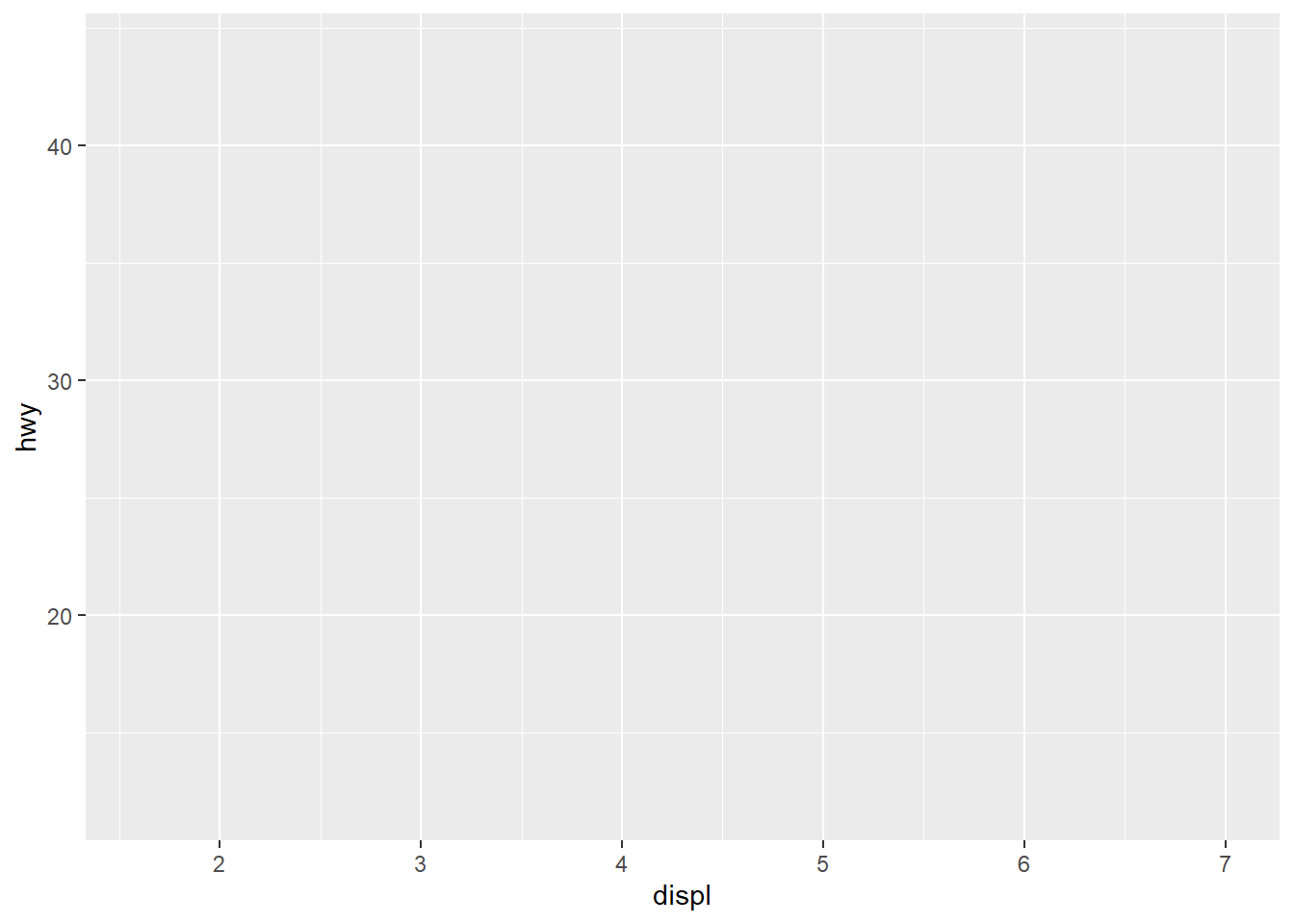ggplot(data = mpg) +
geom_point(mapping = aes(x = displ,
y = hwy))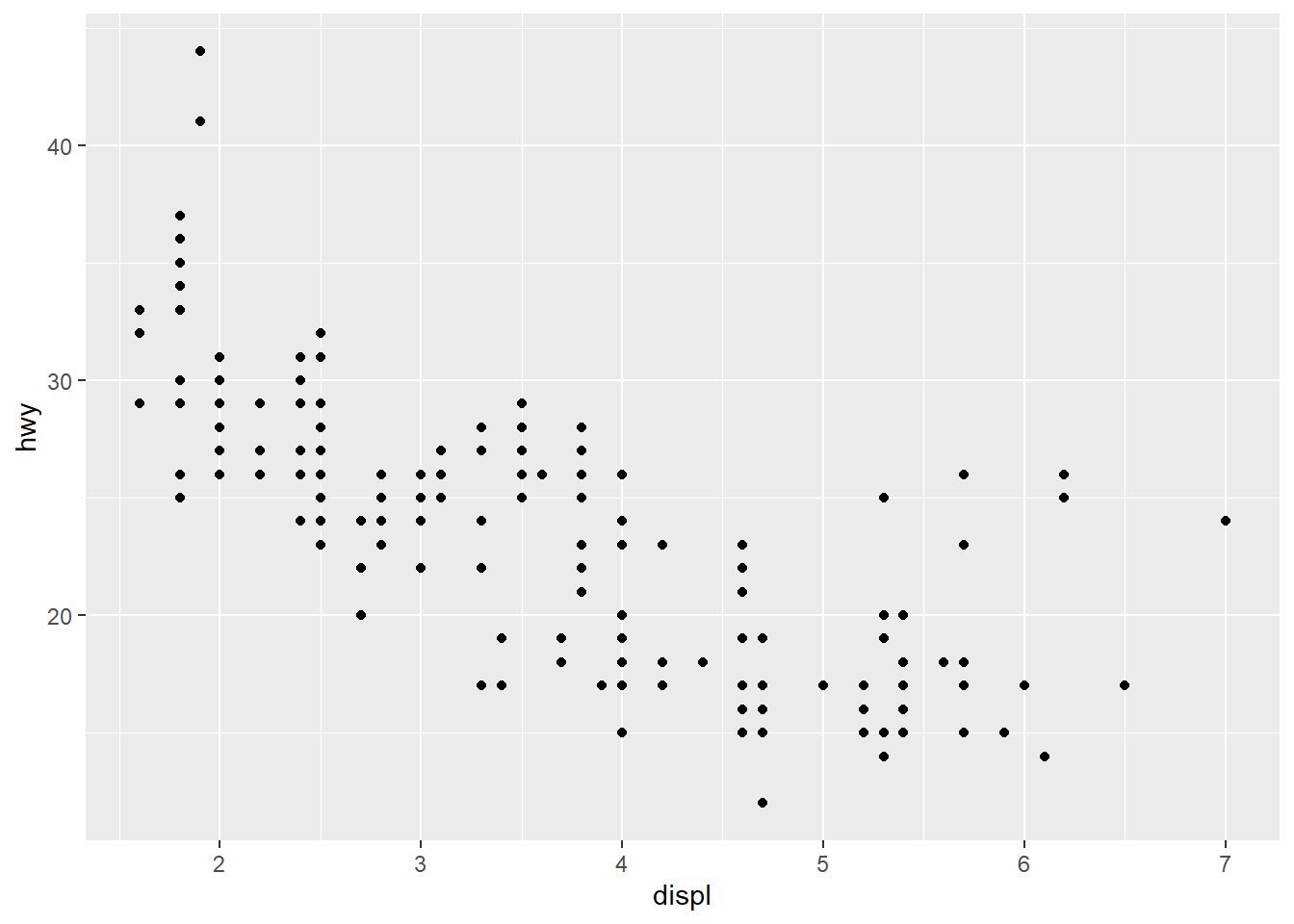My hypothesis has been confirmed.

num_rows <- nrow(mtcars)
num_cols <- ncol(mtcars)
ex4_plot <- ggplot(data = mpg,
aes(x = hwy,
y = cyl)) +
geom_point()

### Exercises

1. Run ggplot(data = mpg). What do you see?
Ans: An empty canvas of a plot. If you add the aes(x = xx, y = yy) you will see an empty canvas with the axes drawn.
2. How many rows are there in mtcars? Columns?
Ans: Number of rows is 32, cols is 11.
3. What does the drv variable describe? Ans: ‘The type of drive train, where f = front-wheel drive, r = rear wheel drive, 4 = 4wd’
4. Make a scatterplot of hwy versus cyl.
ex4_plot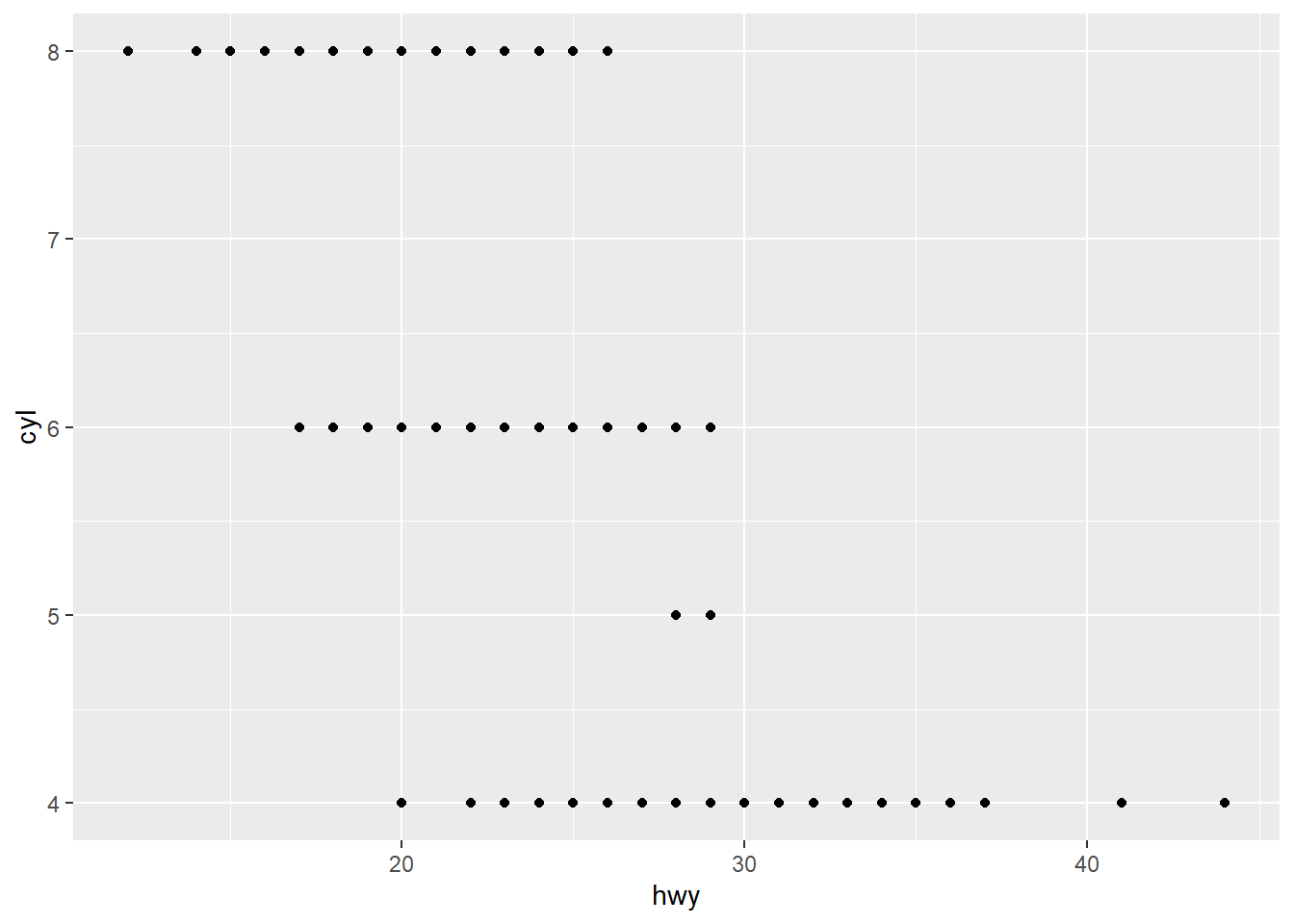1. What happens if you make a scatterplot of class versus drv? Why is this plot not useful?
ggplot(data = mpg, aes(x = class,
y = drv)) +
geom_point()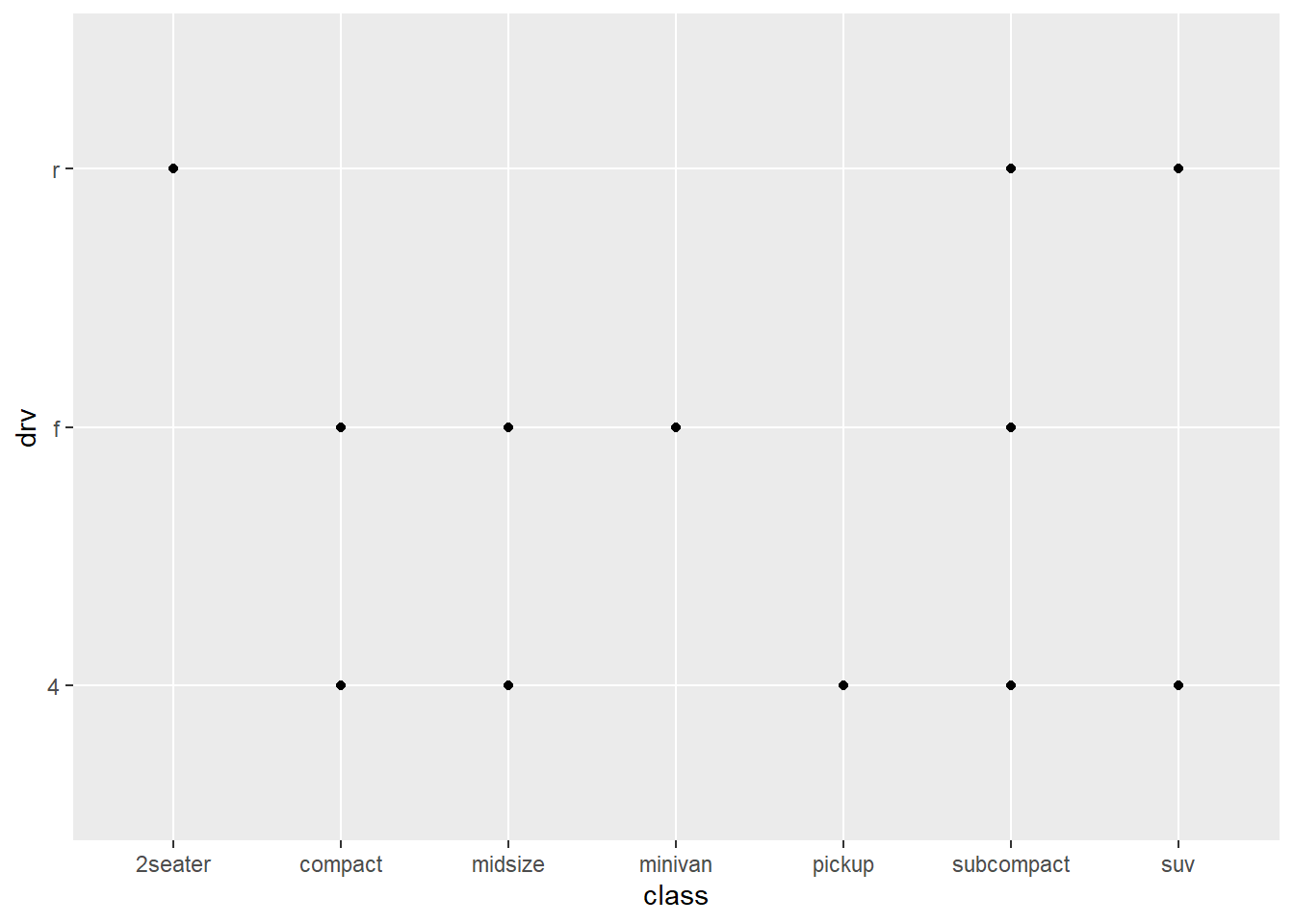These are 2 categorical variables here so this isn’t very useful.

## Aesthetics

You can give extra information about your dataset by mapping data to aesthetics like size, colour, shape.

ggplot(data = mpg) +  geom_point(mapping = aes(x = displ, y = hwy,                            colour = class))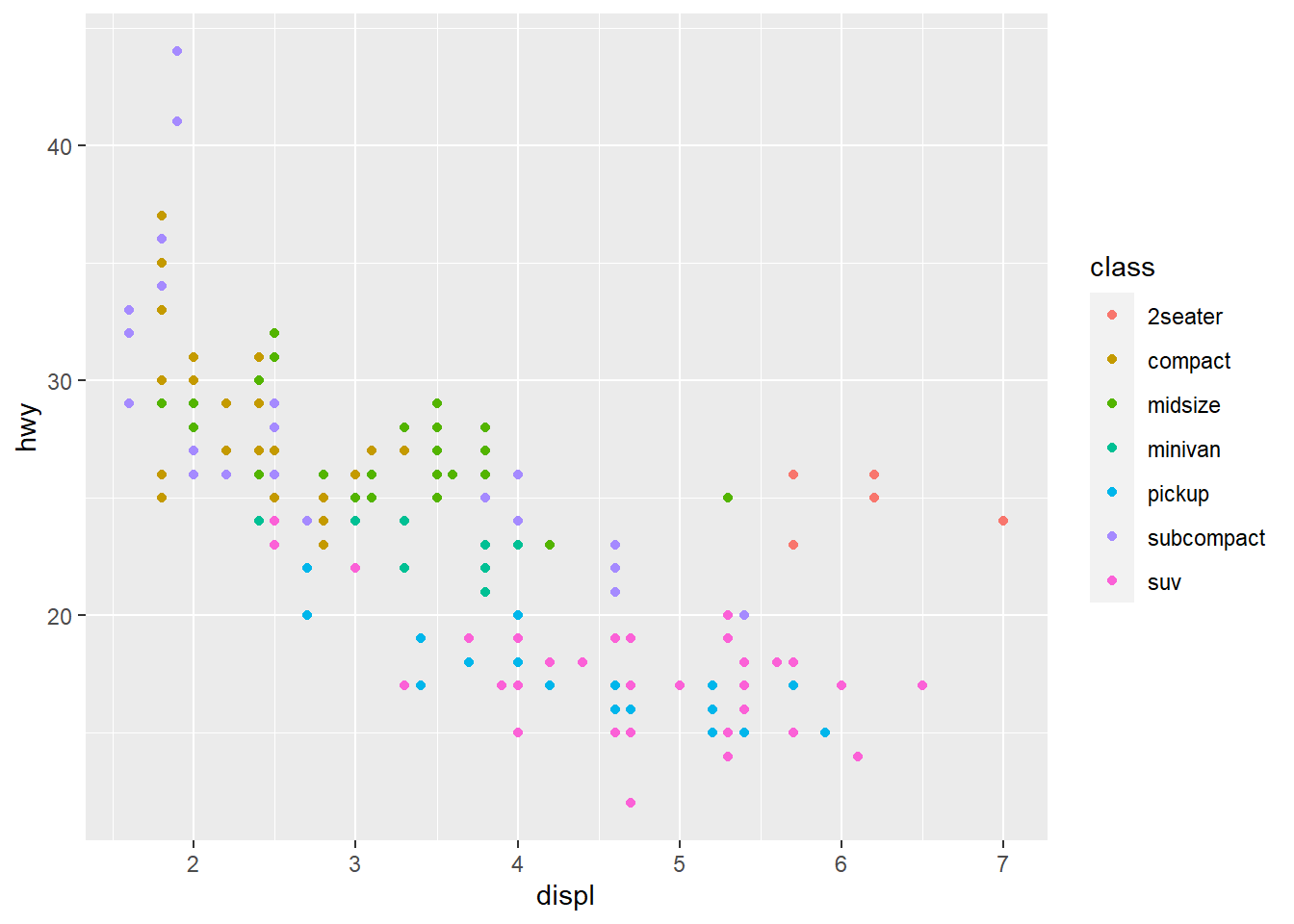When you put a feature against an aesthetic ggplot will assign a unique level (here colour to each class of the feature) -> this process is called scaling.

ggplot(data = mpg) +  geom_point(aes(x = displ, y = hwy,                 size = class))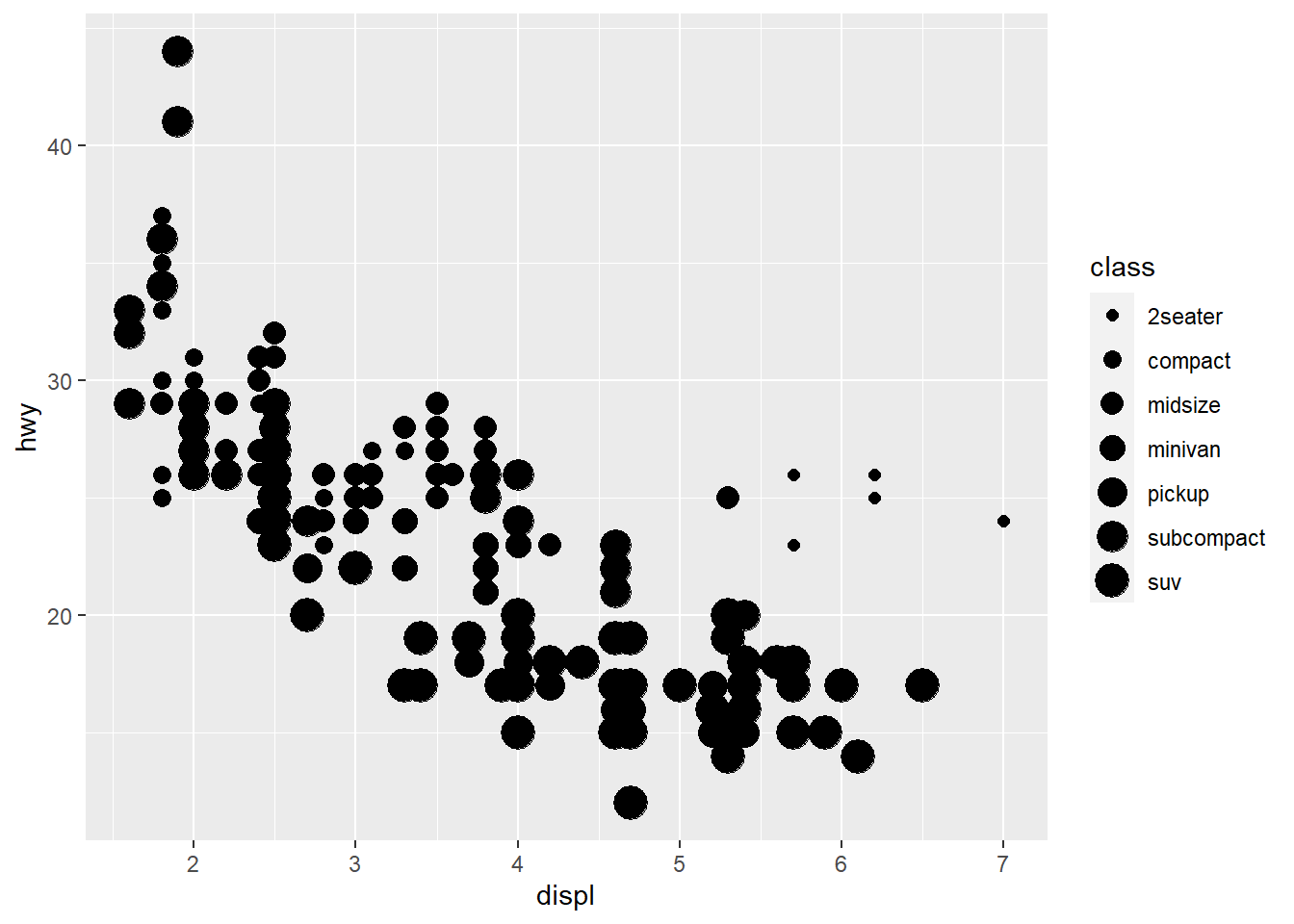Mapping a unordered variable like class to an ordered aesthetic like size is not a good idea, and we get a warning here.

ggplot(data = mpg) +  geom_point(aes(x = displ, y = hwy,                 alpha = class))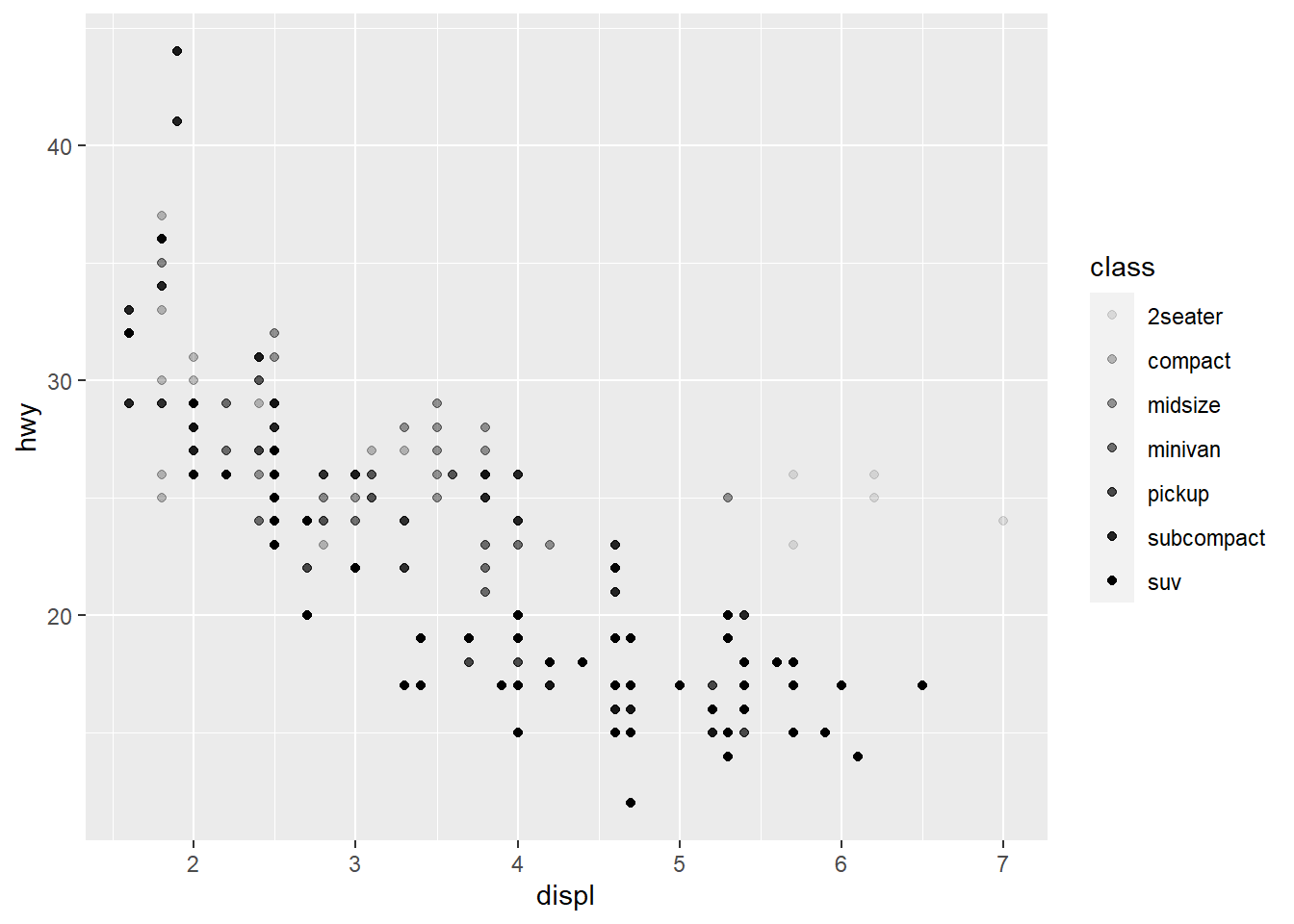alpha is another aesthetic that we can use.

ggplot(data = mpg) +  geom_point(aes(x = displ, y = hwy,                 shape = class))

Warning: The shape palette can deal with a maximum of 6 discrete values because
more than 6 becomes difficult to discriminate; you have 7. Consider
specifying shapes manually if you must have them.


Warning: Removed 62 rows containing missing values (geom_point).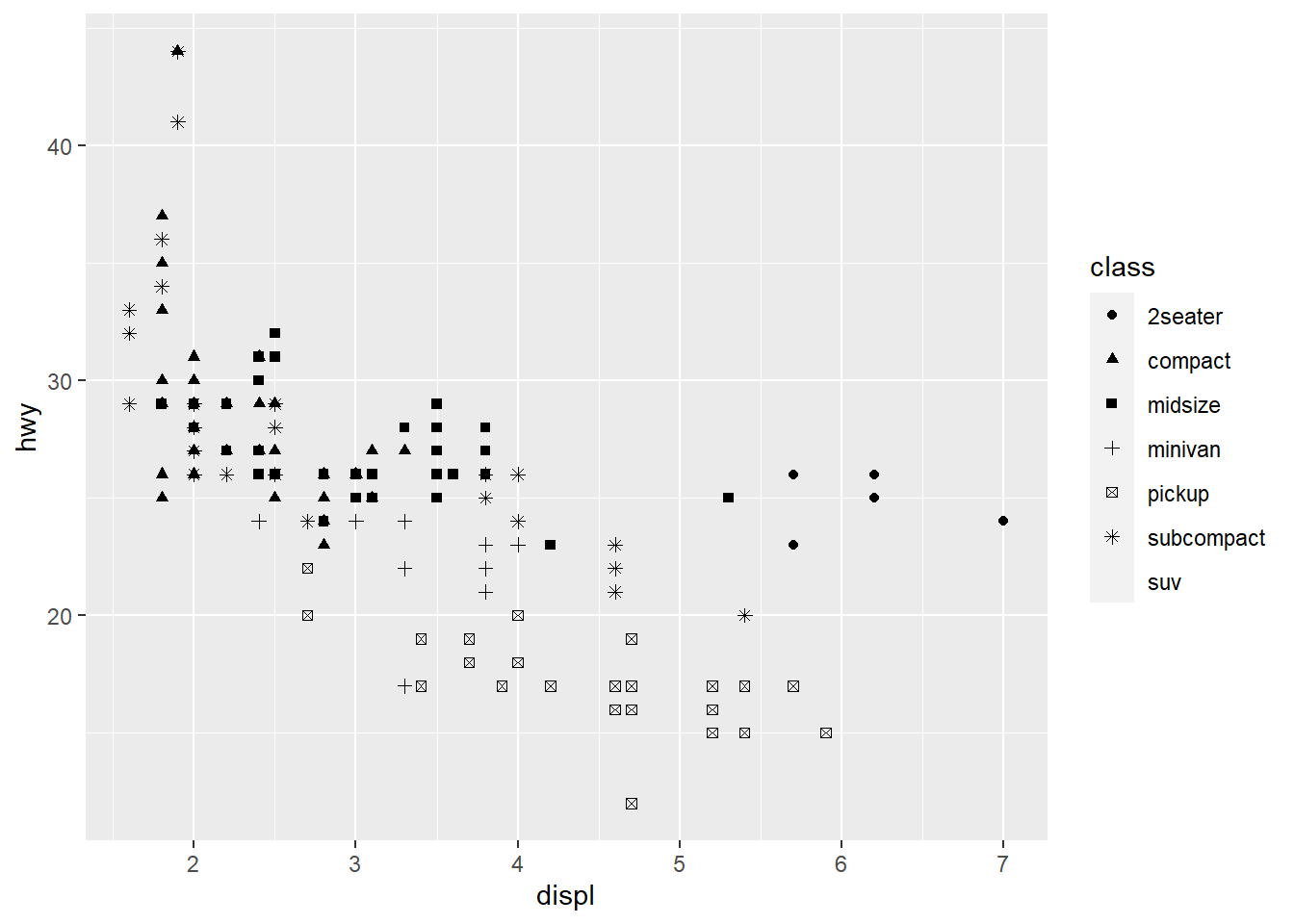ggplot only uses 6 shapes at a time, so we’re missing suv! When mapping to aesthetics think carefully about which aesthetic makes sense.

If you look at the colour = class plot vs the shape = class plot you may well think - it looks like previously labelled suv is now being considered to be pickup!? There are many points and the points get plotted on top of each other - if you look near displ = 5 and hwy = 12 notice that there is an pickup point there … previously there was an suv point there. Let’s jitter the data so that the points lie a little away from each other to show that there’s not any issue with ggplot, instead the data lying on top of each other shows the last class.

ggplot(data = mpg) +
geom_point(aes(x = displ, y = hwy,
colour = class))ggplot(data = mpg) +
geom_point(aes(x = displ, y = hwy,
shape = class))ggplot(data = mpg) +
geom_jitter(aes(x = displ, y = hwy,
colour = class))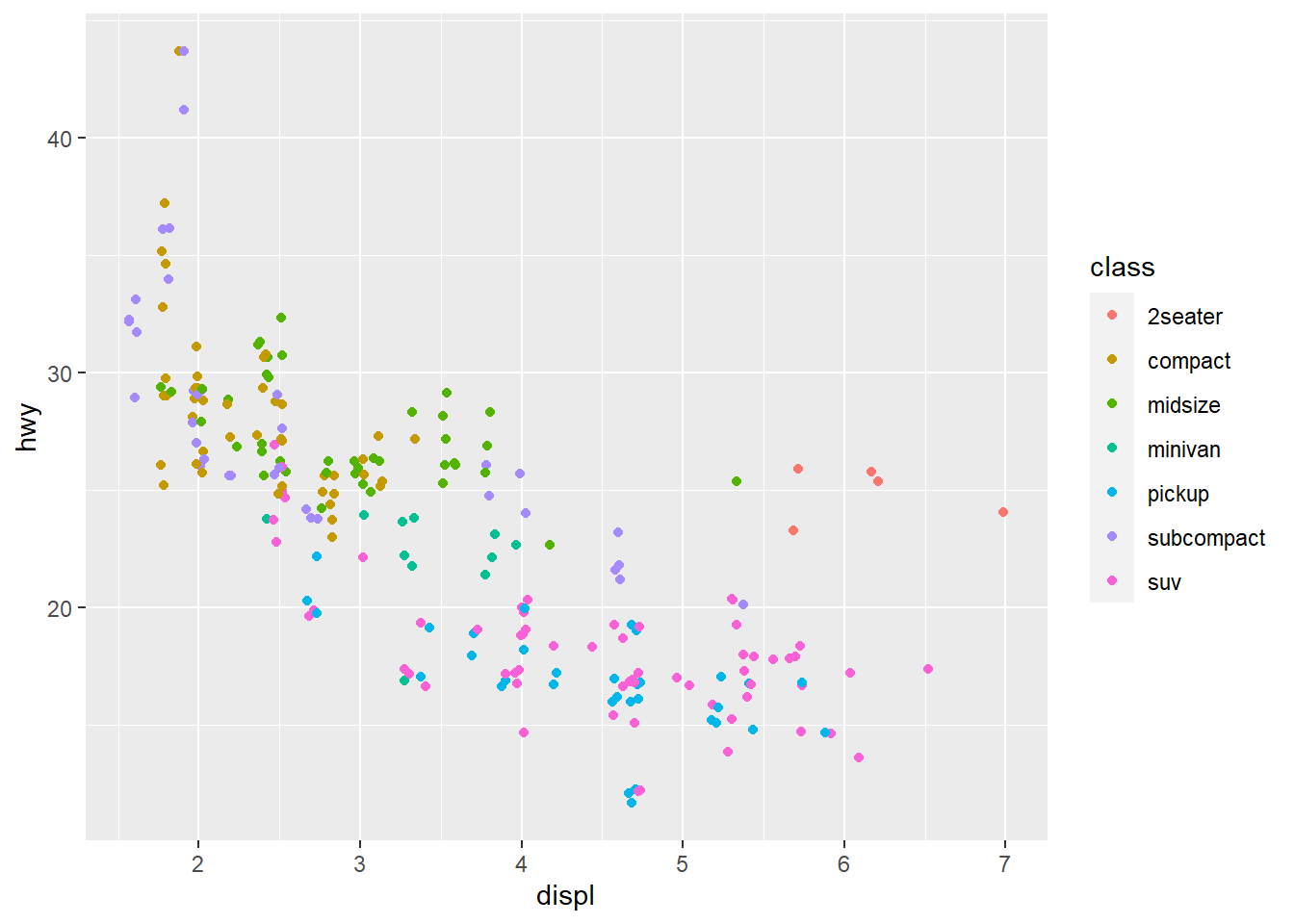ggplot(data = mpg) +
geom_jitter(aes(x = displ, y = hwy,
shape = class))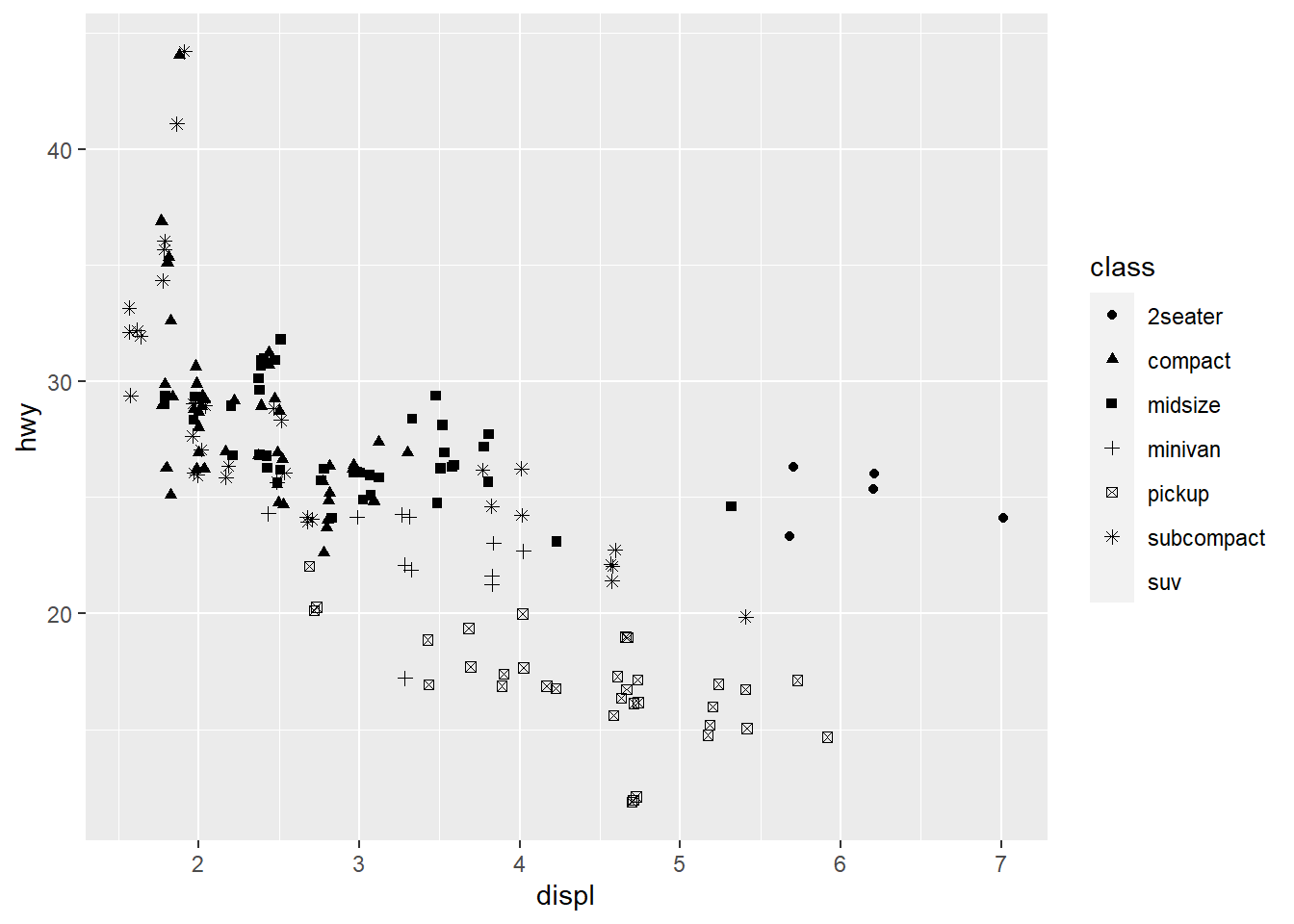Occassionally you may want to change all the points sizes uniformly (irrespective of any other data feature) - in this case you may put the aesthetic on the outside of the aes().

ggplot(data = mpg) +  geom_point(aes(x = displ, y = hwy),             colour = "blue")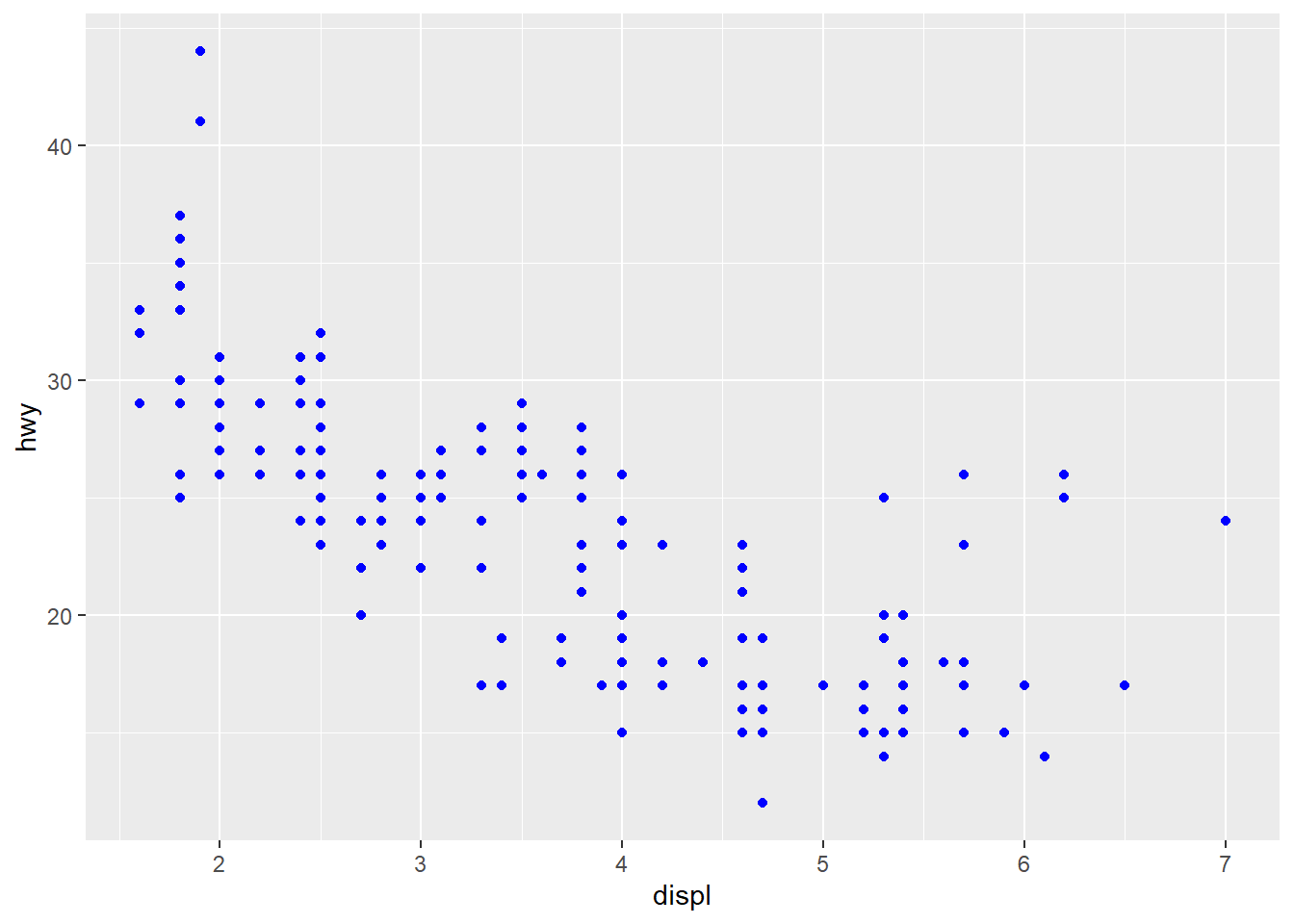ggplot(data = mpg) +  geom_point(aes(x = displ,                  y = hwy),             size = 4)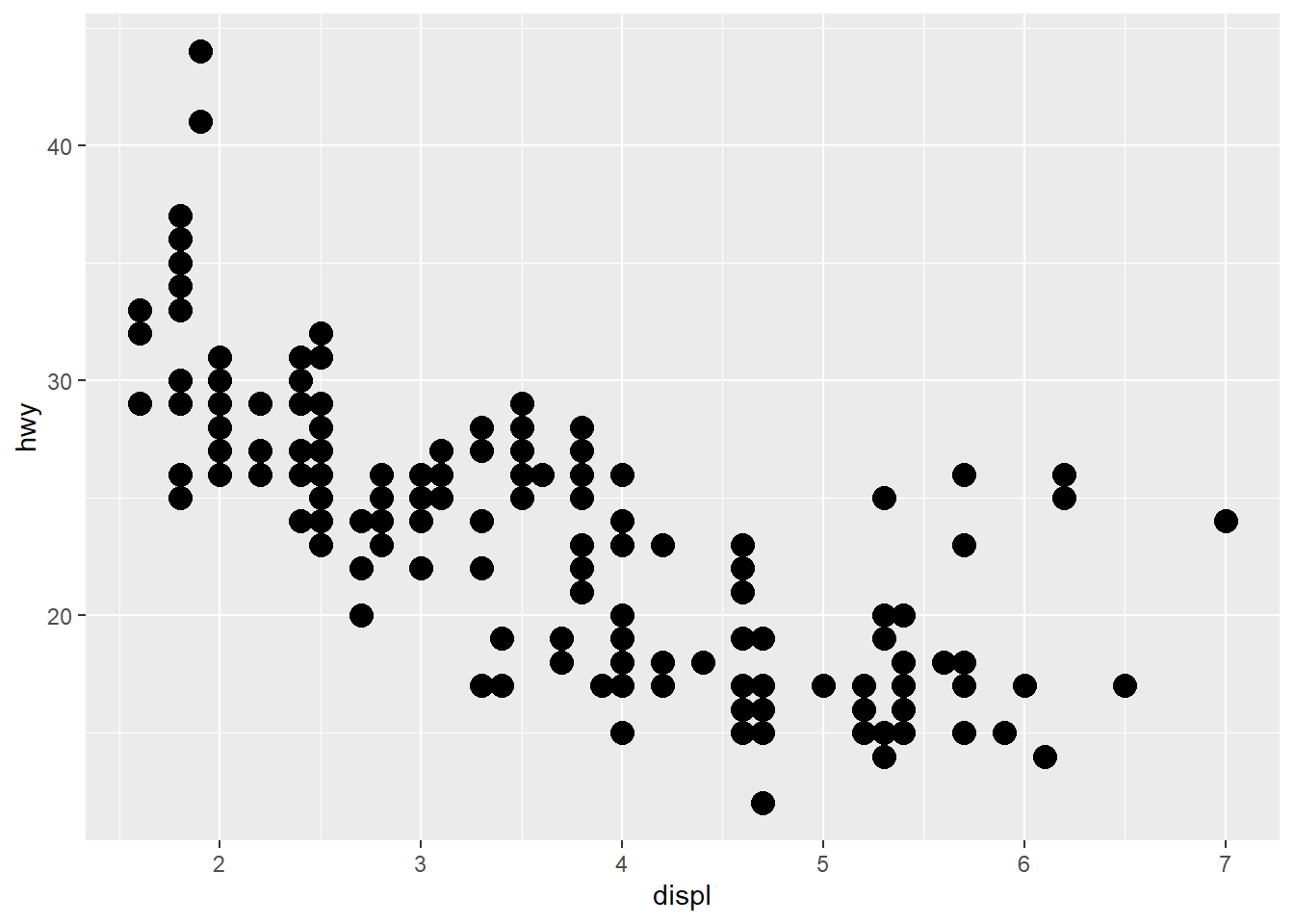### Exercises

1. What’s gone wrong with this code? Why are the points not blue?
ggplot(data = mpg) +   geom_point(mapping = aes(x = displ,                            y = hwy, color = "blue"))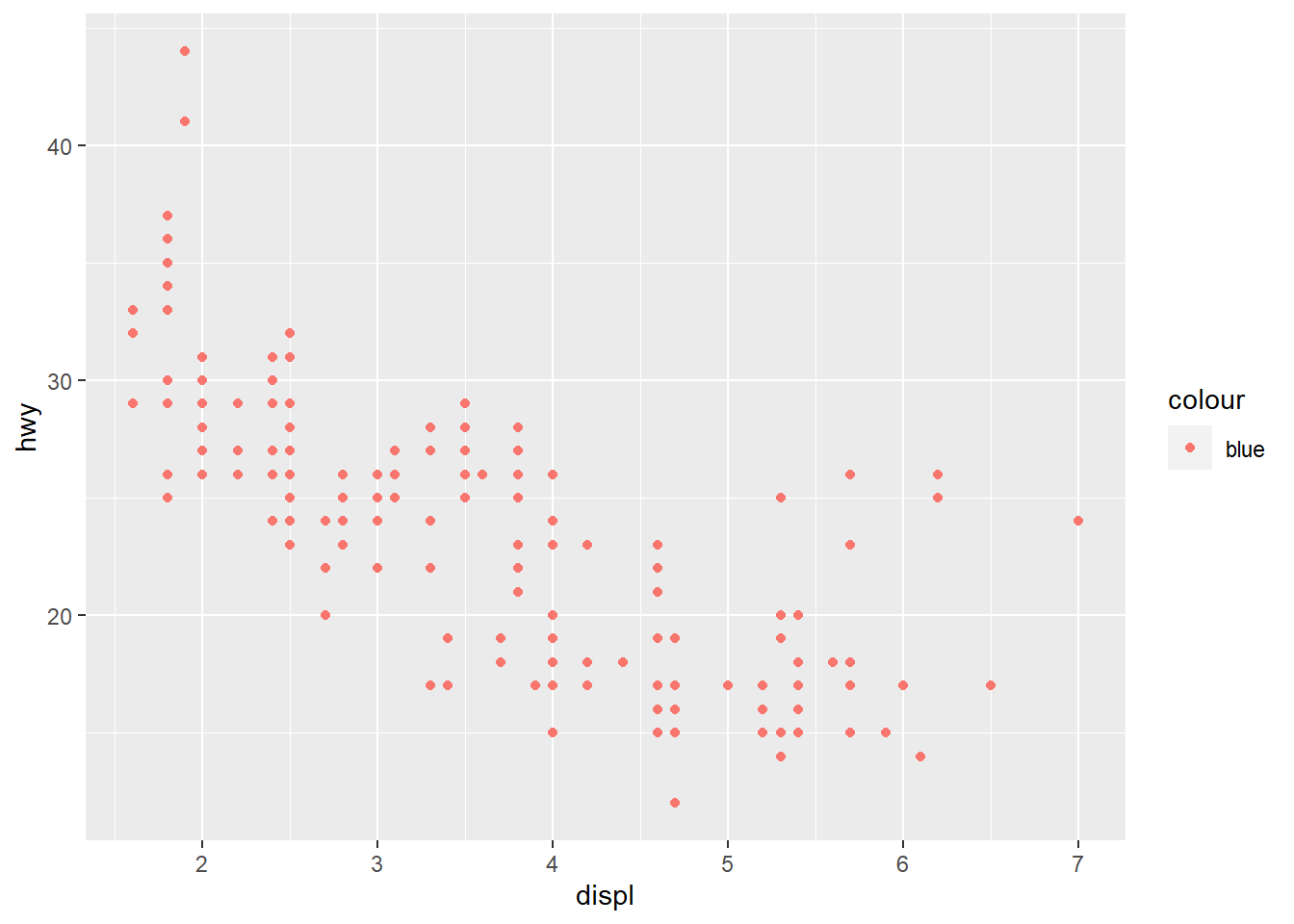The color attribute maps to a static colour and yet appears within aes().

To correct, put colour = 'blue' outside the aes()

ggplot(data = mpg) +   geom_point(mapping = aes(x = displ,                            y = hwy),              color = "blue")1. Which variables in mpg are categorical? Which variables are continuous? (Hint: type ?mpg to read the documentation for the dataset). How can you see this information when you run mpg?

manufacturer, model, trans, drv, fl and class are categories (I also think cyl may be considered a category since it takes on 4 values based on a limited number of cylinders); displ, year, cty, hwy, cyl are continuous. You can convert mpg to a tibble (if it is not already) and check the types using glimpse().

glimpse(mpg)
Rows: 234
Columns: 11
$manufacturer <chr> "audi", "audi", "audi", "audi", "audi", "audi", "audi"...$ model        <chr> "a4", "a4", "a4", "a4", "a4", "a4", "a4", "a4 quattro"...
$displ <dbl> 1.8, 1.8, 2.0, 2.0, 2.8, 2.8, 3.1, 1.8, 1.8, 2.0, 2.0,...$ year         <int> 1999, 1999, 2008, 2008, 1999, 1999, 2008, 1999, 1999, ...
$cyl <int> 4, 4, 4, 4, 6, 6, 6, 4, 4, 4, 4, 6, 6, 6, 6, 6, 6, 8, ...$ trans        <chr> "auto(l5)", "manual(m5)", "manual(m6)", "auto(av)", "a...
$drv <chr> "f", "f", "f", "f", "f", "f", "f", "4", "4", "4", "4",...$ cty          <int> 18, 21, 20, 21, 16, 18, 18, 18, 16, 20, 19, 15, 17, 17...
$hwy <int> 29, 29, 31, 30, 26, 26, 27, 26, 25, 28, 27, 25, 25, 25...$ fl           <chr> "p", "p", "p", "p", "p", "p", "p", "p", "p", "p", "p",...
\$ class        <chr> "compact", "compact", "compact", "compact", "compact",...
1. Map a continuous variable to color, size, and shape. How do these aesthetics behave differently for categorical vs. continuous variables?
ggplot(data = mpg) +
geom_point(aes(x = displ, y = hwy,
colour = cyl))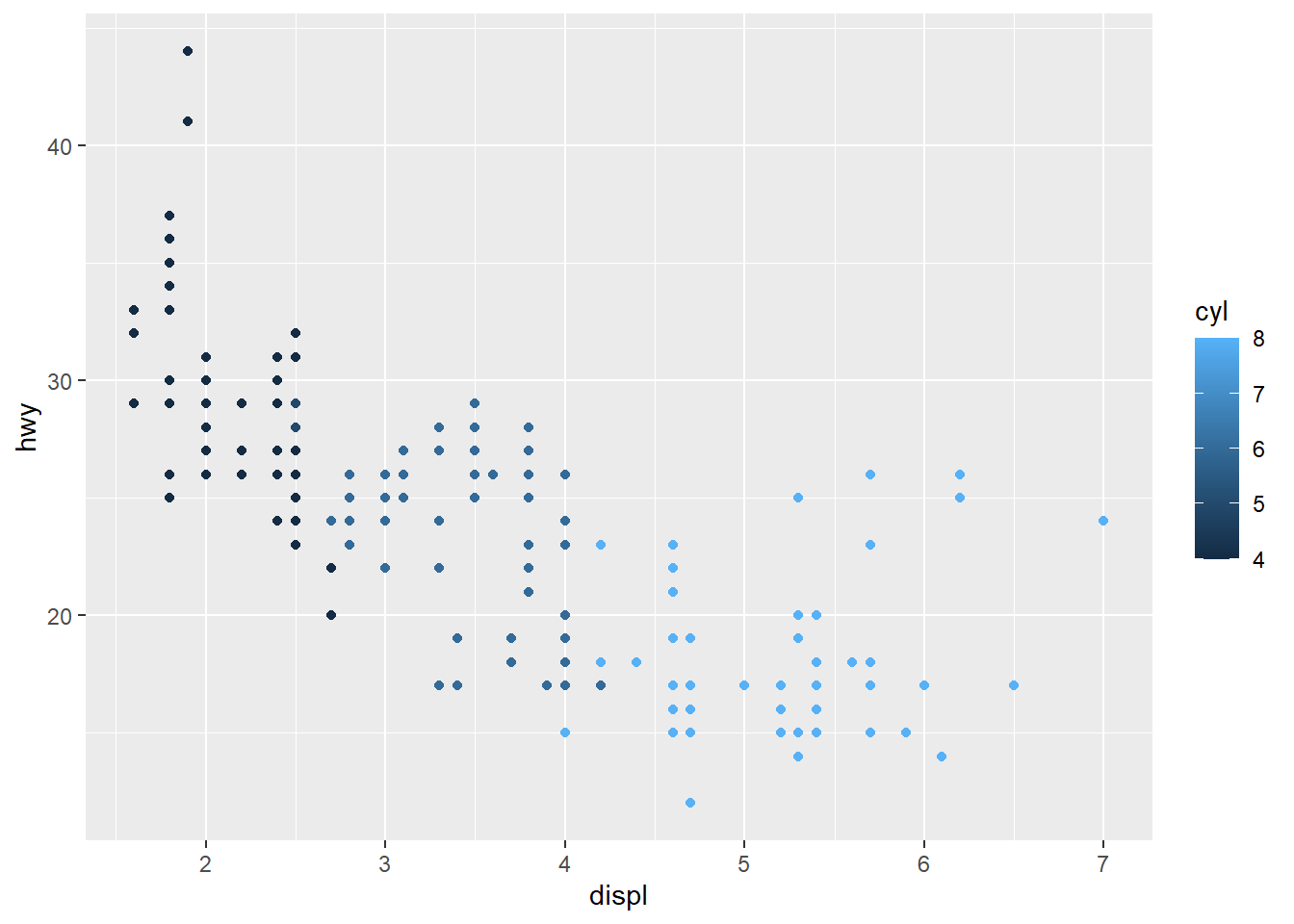ggplot(data = mpg) +
geom_point(aes(x = displ, y = hwy,
colour = cty))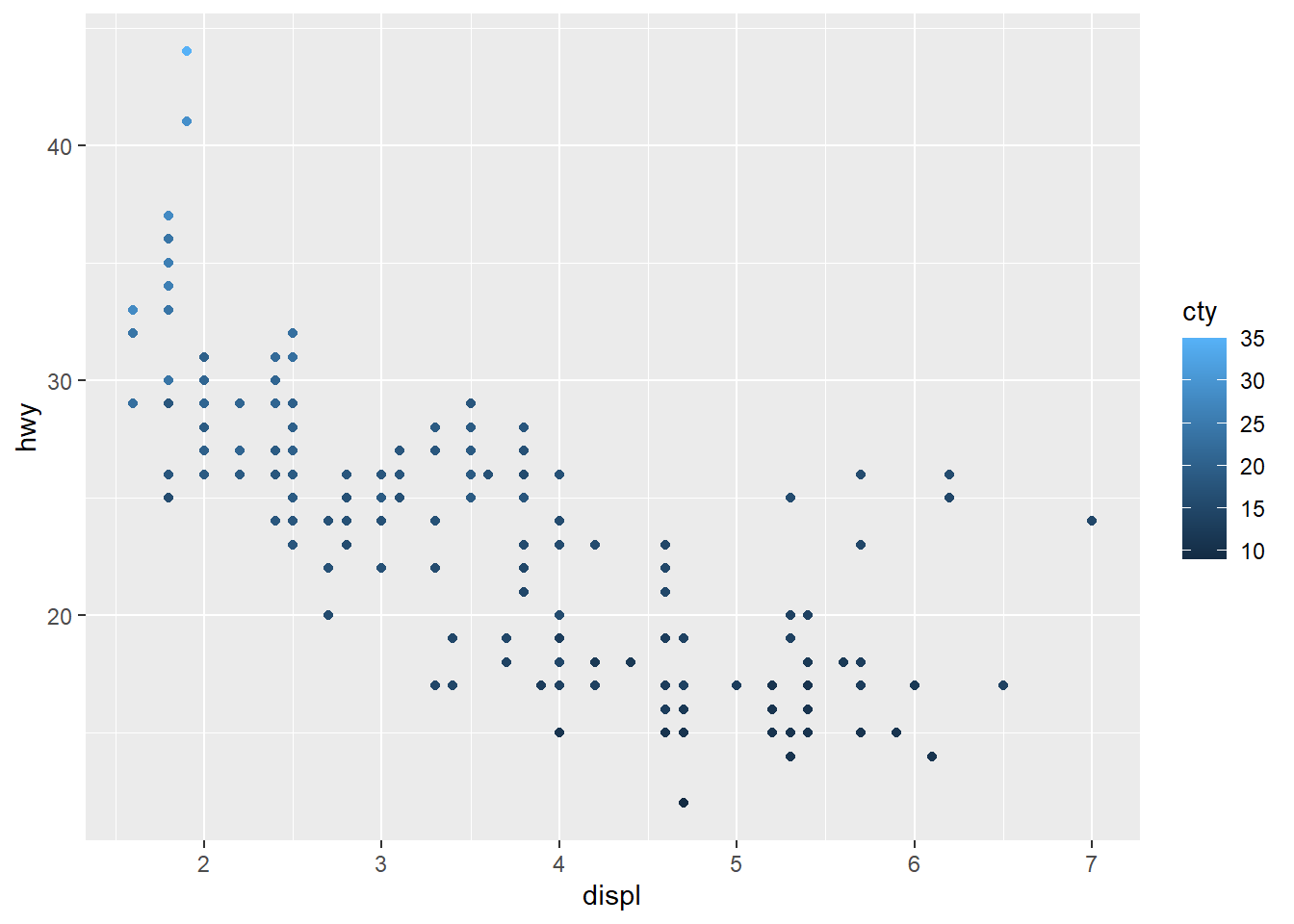The colours are scaled from the lowest to the highest value using dark to light blue.

1. What happens if you map the same variable to multiple aesthetics?
ggplot(data = mpg) +  geom_point(aes(x = displ, y = hwy,                 colour = class,                 size = class))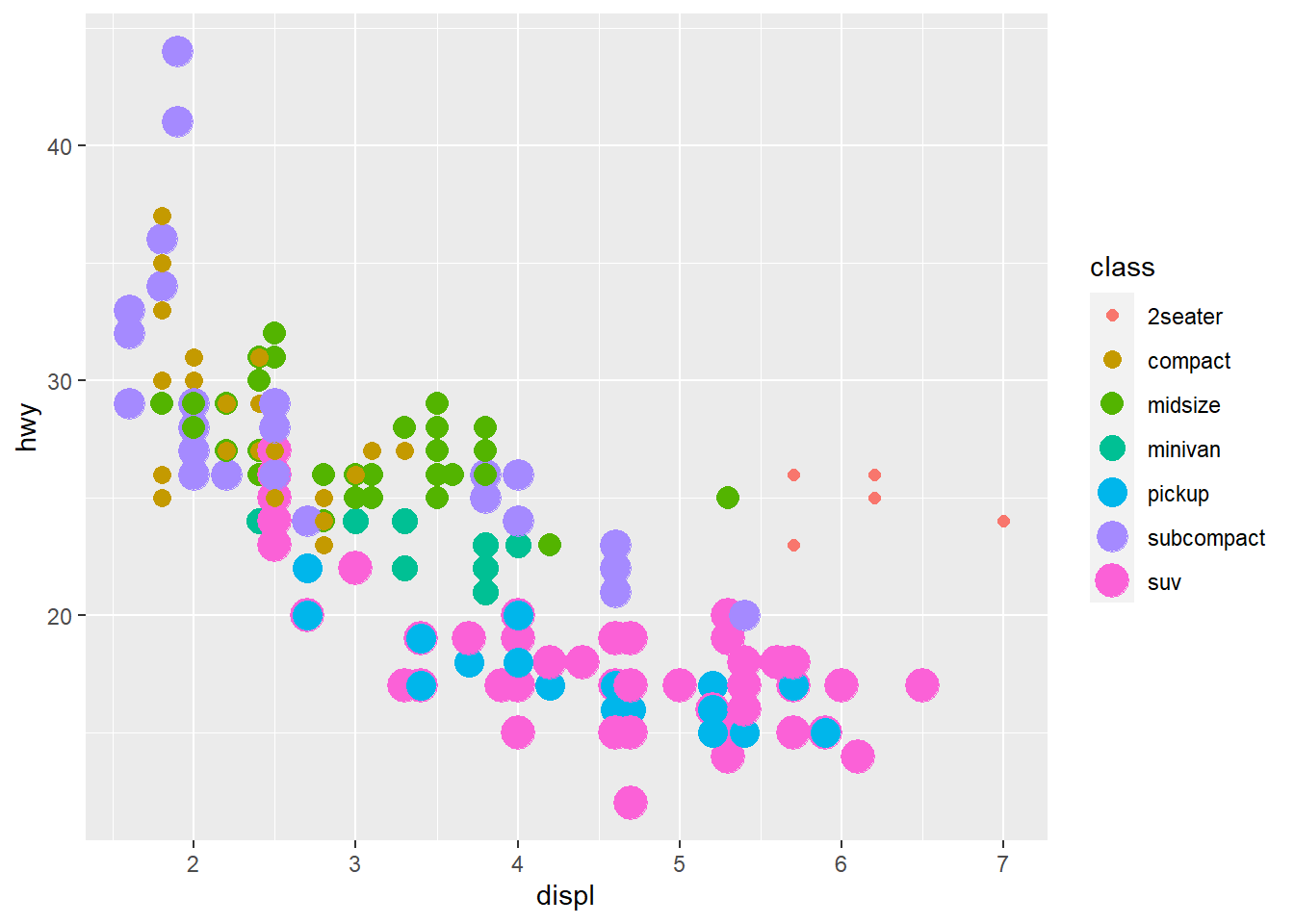Here we have both colour and size mapped to class. The plot offers no new information with the second aesthetic so I’d consider it a poor plot. You should use aesthetics mapped to different features in your data to meet with the Axiom that ‘The greatest value of a picture is when it forces us to notice what we never expected to see. –John Tukey’ as mentioned in the beginning of the Aesthetic Mappings section!

1. What does the stroke aesthetic do? What shapes does it work with? (Hint: use ?geom_point)

Tip: Did you know that when you call ?geom_point you can go to an example and highlight it, press ‘Ctrl + Enter’ and it will place that example in your console and run it?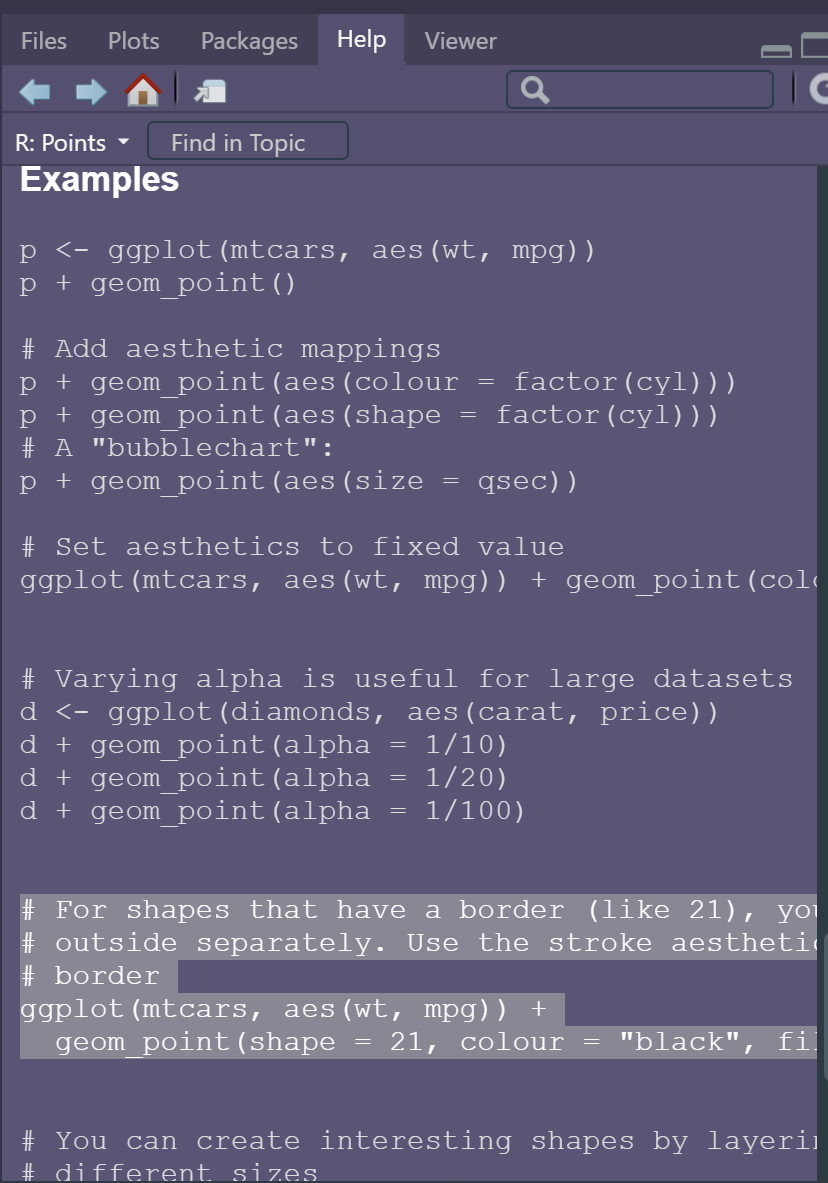# For shapes that have a border (like 21), you can colour the inside and
# outside separately. Use the stroke aesthetic to modify the width of the
# border
ggplot(mtcars, aes(wt, mpg)) +
geom_point(shape = 2, colour = "black", fill = "white", size = 5, stroke = 5)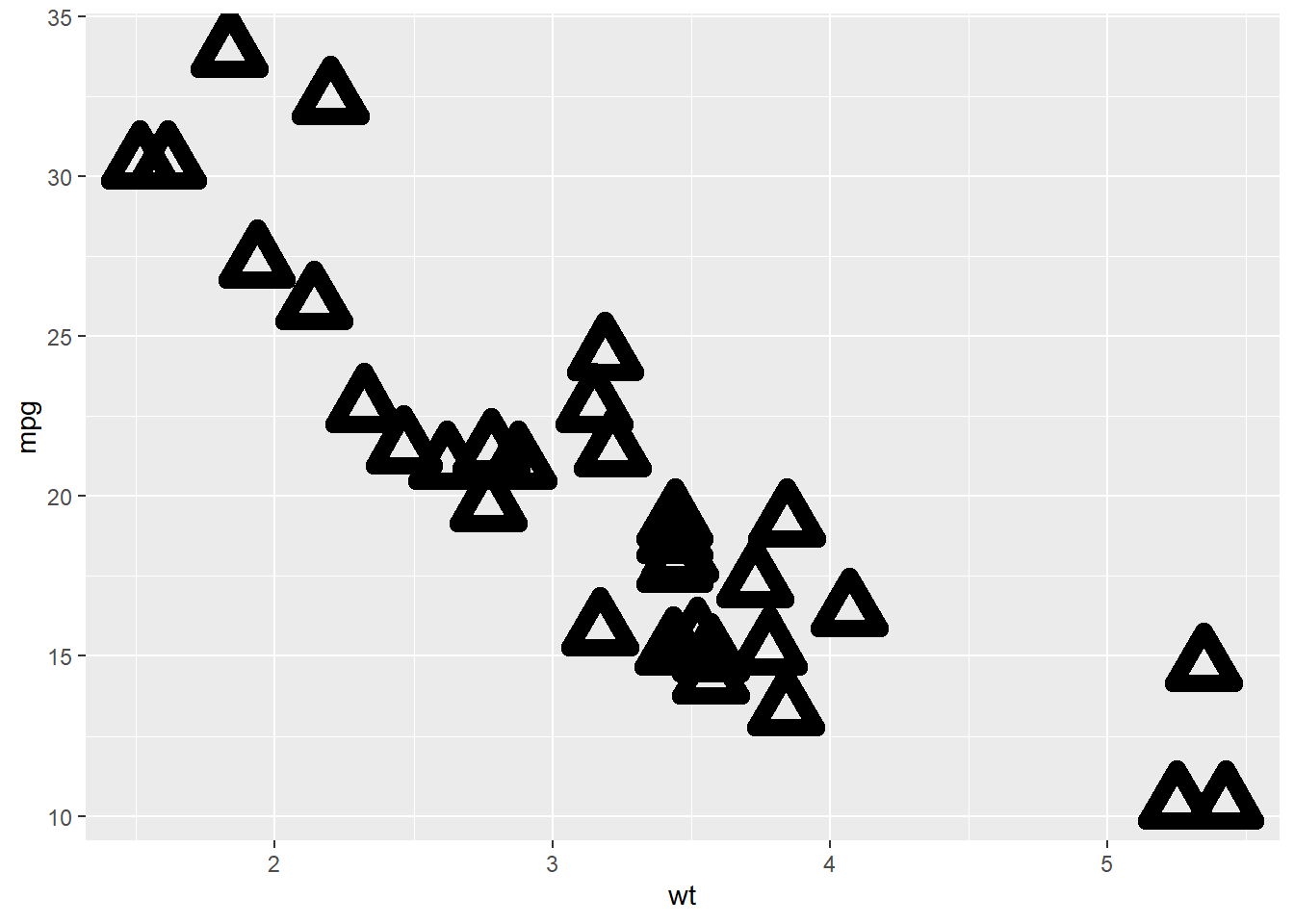ggplot(mtcars, aes(wt, mpg)) +
geom_point(shape = 23, colour = "black", fill = "white", size = 5, stroke = 3)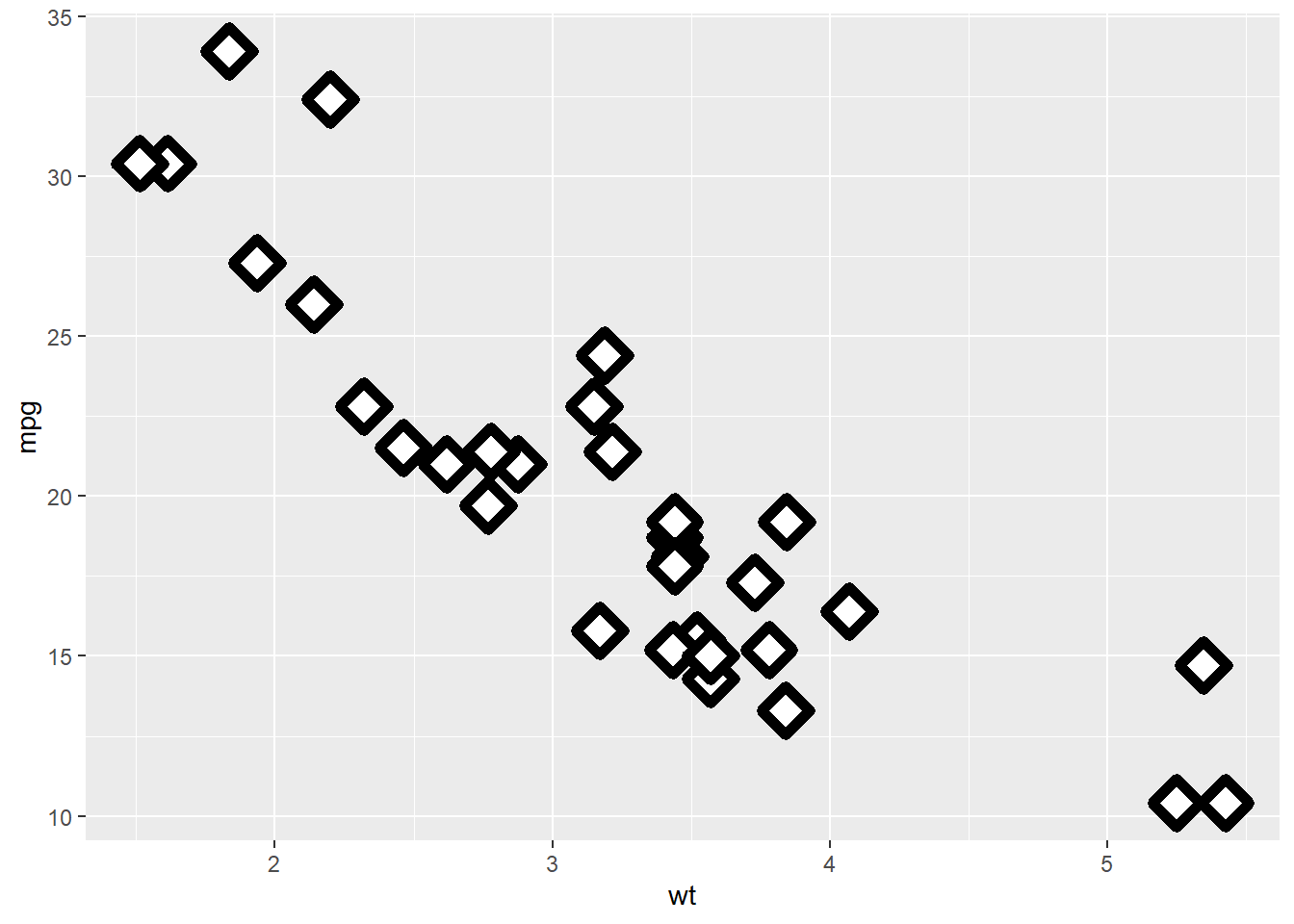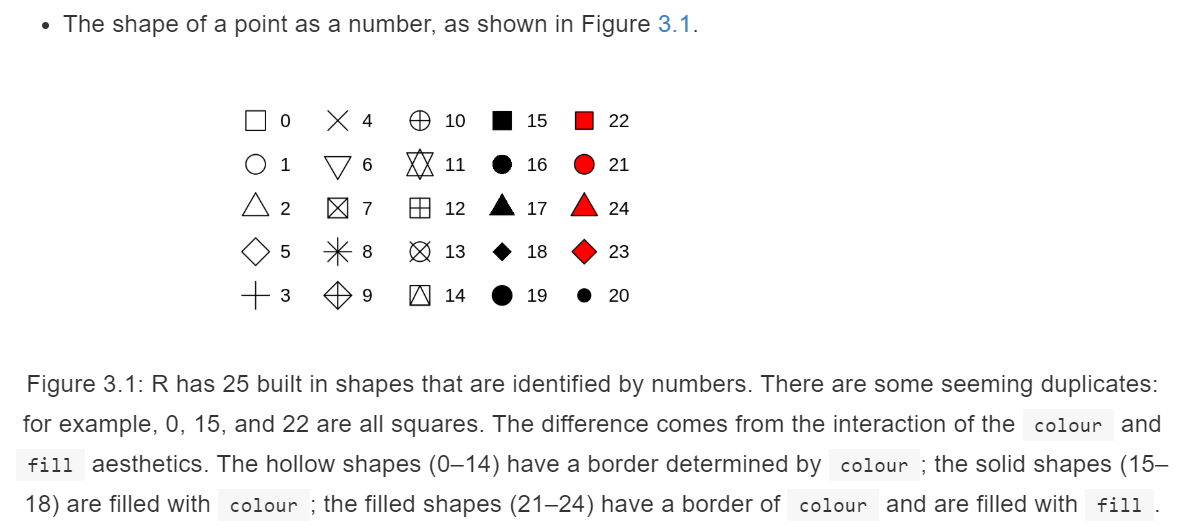Stroke changes the border width as described in the example comment, and works with any shape that has a border hence will work with shapes 0-14, and 21-24. An example of each is above.

1. What happens if you map an aesthetic to something other than a variable name, like aes(colour = displ < 5)? Note, you’ll also need to specify x and y.
ggplot(data = mpg) +   geom_point(mapping = aes(x = displ, y = hwy,             colour = displ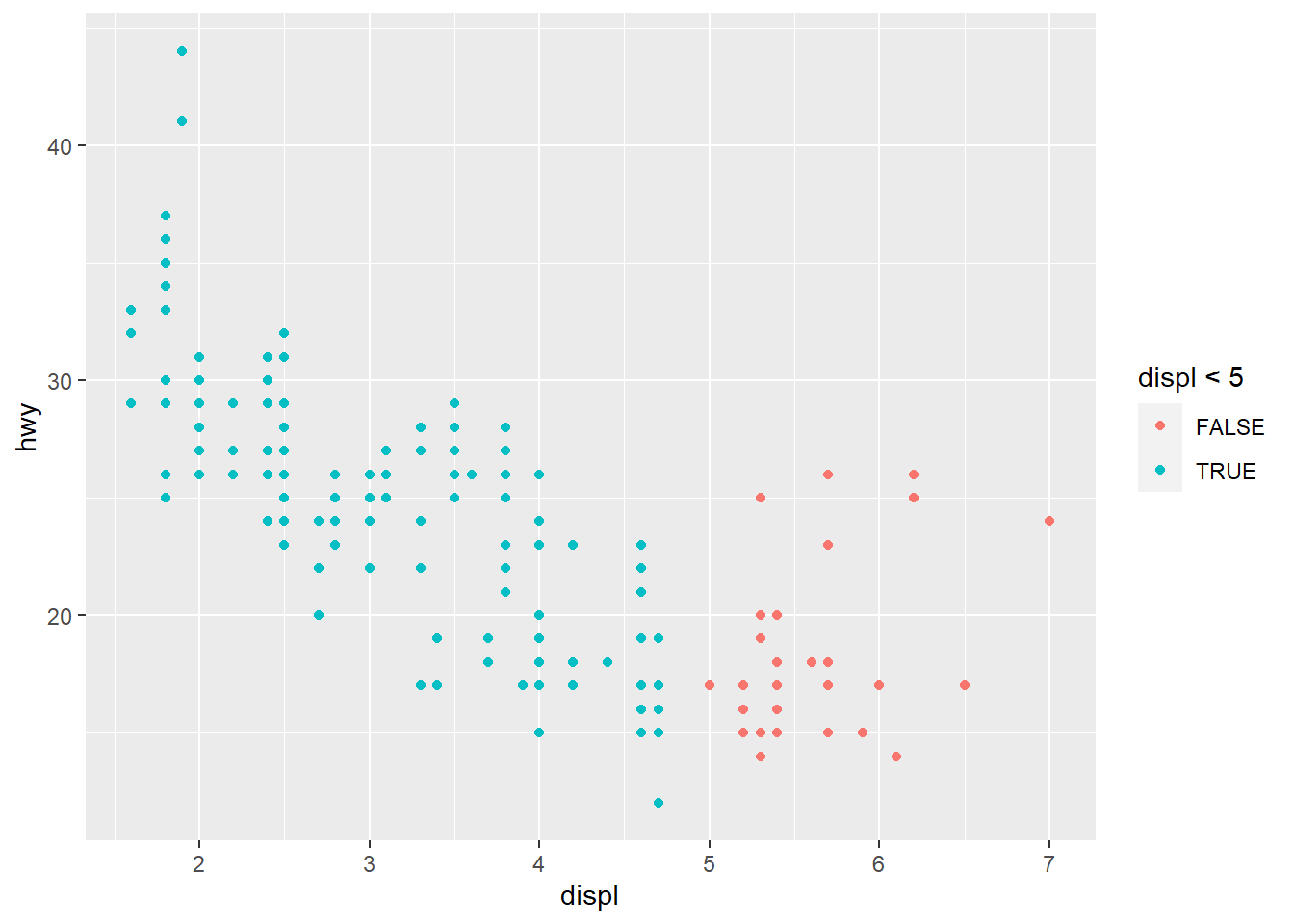Points are split according to the criteria displ < 5 and are coloured differently depending on what value they have for displacement.

## Facets

Another way to add information on your plot is to use facets.

• facet_wrap() allows faceting by a single variable

• First argument is a formula ~ var_name
• Variable passed in should be discrete.
• facet_grid() allows the faceting by 2 variables.

• var_name1 ~ var_name2
ggplot(data = mpg) +  geom_point(mapping = aes(x = displ,                           y = hwy)) +  facet_wrap(~ class, nrow = 2)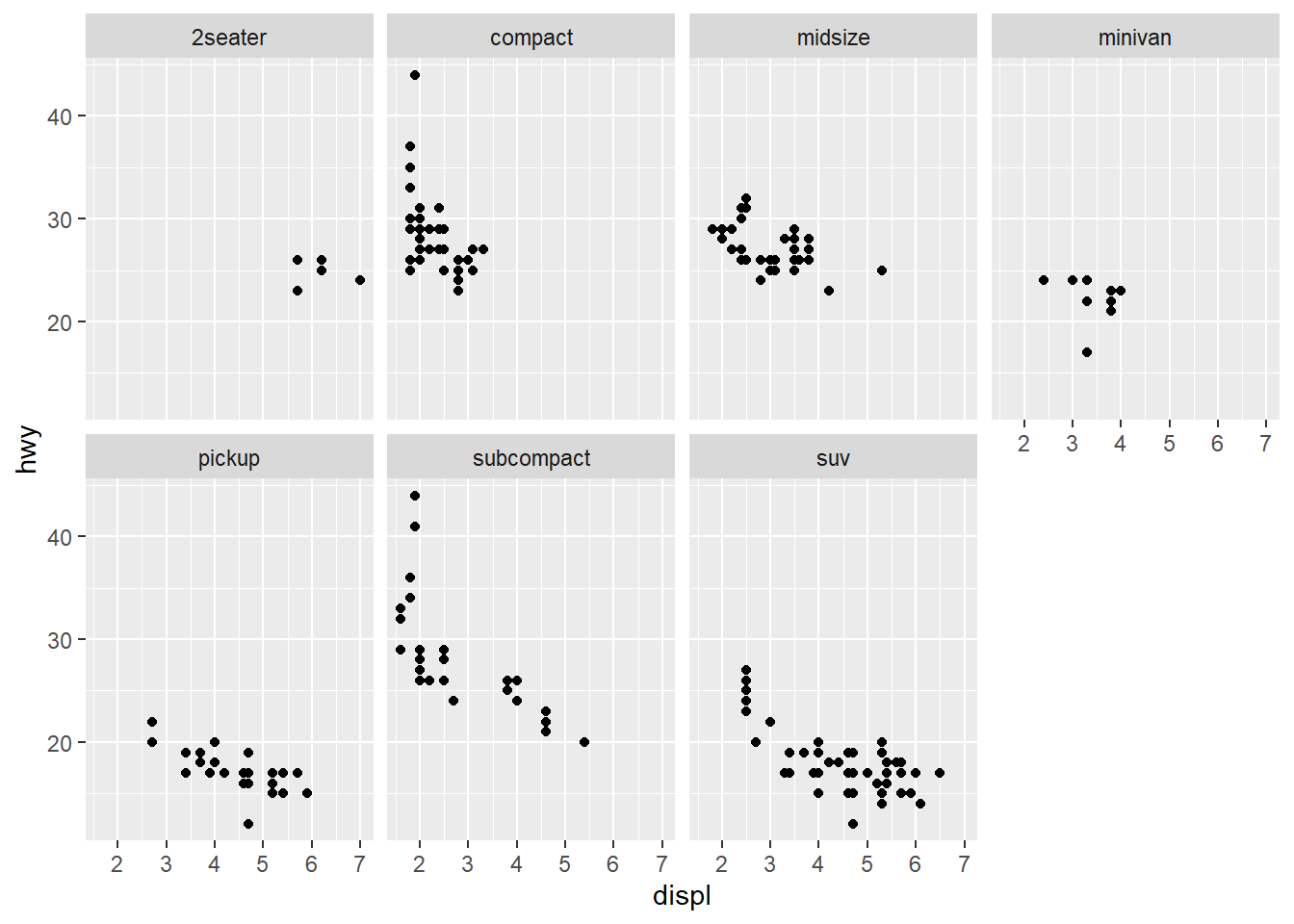ggplot(data = mpg) +  geom_point(mapping = aes(x = displ,                           y = hwy)) +  facet_grid(drv ~ class)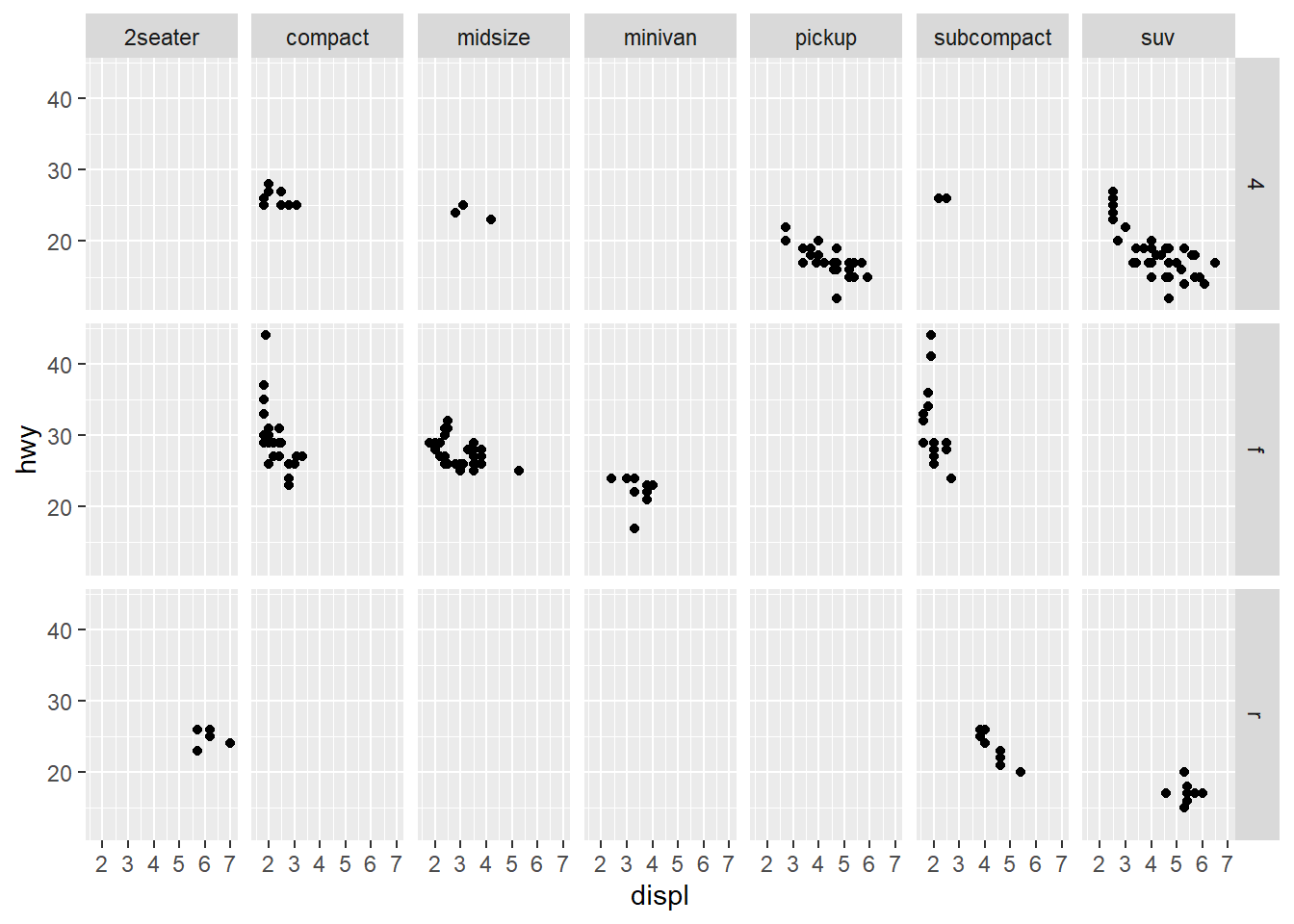### Exercises

1. What happens if you facet on a continuous variable?
ggplot(data = mpg) +  geom_point(aes(x = displ,                 y = hwy)) +  facet_wrap(~ cty)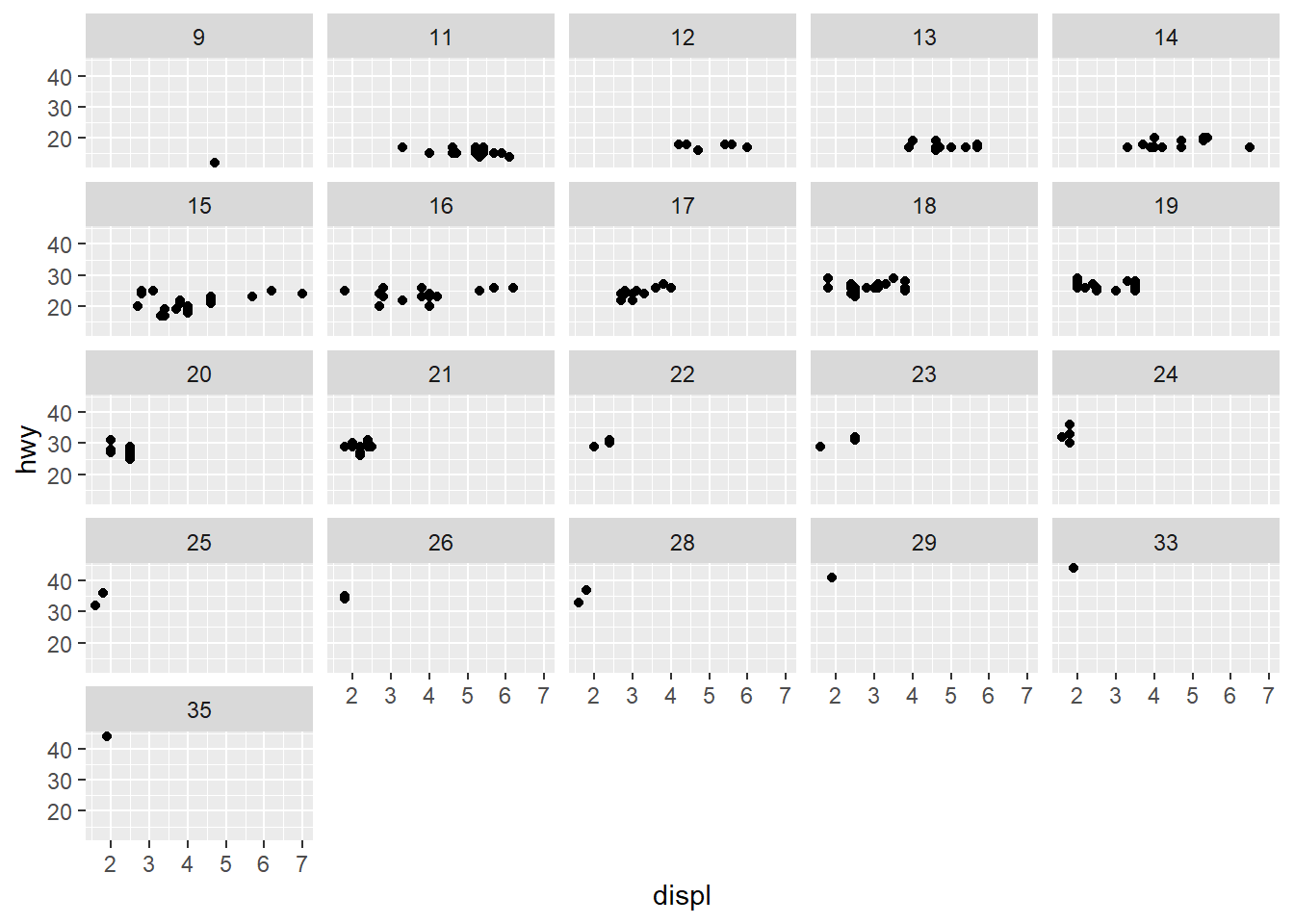The plot is created but it doesn't make sense to split our data
by a continuous variable. 
1. What do the empty cells in plot with facet_grid(drv ~ cyl) mean? How do they relate to this plot?

The empty spots refer to that drv and cyl combination being missing.

ggplot(data = mpg) + <br>
geom_point(mapping = aes(x = drv, y = cyl))

ggplot(data = mpg) +
geom_point(mapping = aes(x = drv, y = cyl))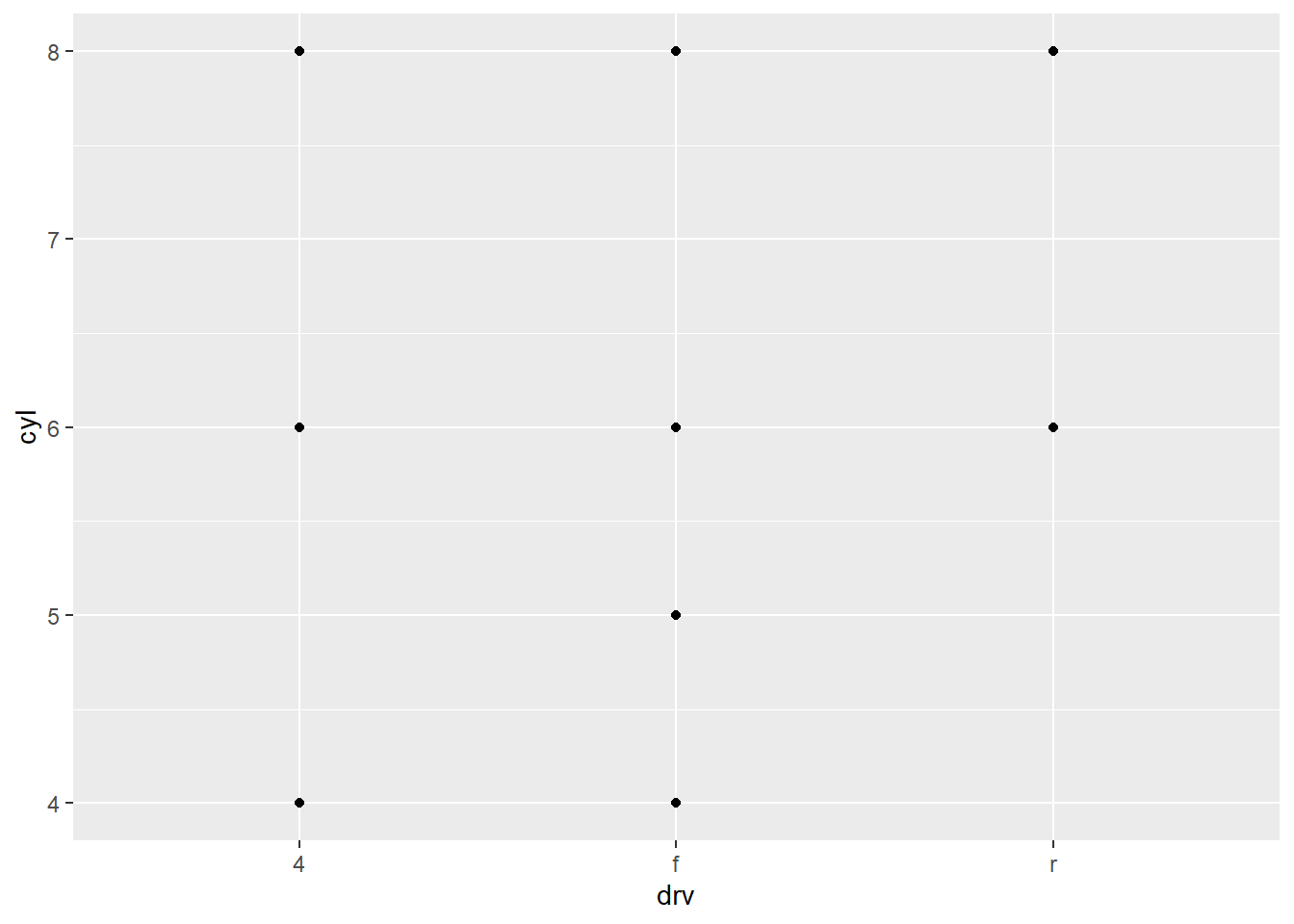It relates to the above in that you can see that the drv = 4; cyl = 5 combination has no observations. This is also the case in the faceted plot.

2. What plots does the following code make? What does . do?

ggplot(data = mpg) +       geom_point(mapping = aes(x = displ, y = hwy)) +      facet_grid(drv ~ .)
 <img src="figure/ch1_ggplot.Rmd/unnamed-chunk-56-1.png" width="672" style="display: block; margin: auto;" />

The facet_grid (drv ~ .) facets the drv categories into rows. It says facet this plot by drv as row panels but I don’t want anything as column panels (hence the .).

ggplot(data = mpg) +       geom_point(mapping = aes(x = displ, y = hwy)) +      facet_grid(. ~ cyl)
 <img src="figure/ch1_ggplot.Rmd/unnamed-chunk-57-1.png" width="672" style="display: block; margin: auto;" />

The facet_grid(. ~ cyl) facets the cyl categories into columns. It says facet this plot by cyl as column panels but I don’t want anything as row panels (hence the .).

3. Take the first faceted plot in this section:

ggplot(data = mpg) +
geom_point(mapping = aes(x = displ, y = hwy)) +
facet_wrap(~ class, nrow = 2)


What are the advantages to using faceting instead of the colour aesthetic? What are the disadvantages? How might the balance change if you had a larger dataset?

ggplot(data = mpg) +
geom_point(mapping = aes(x = displ, y = hwy)) +
facet_wrap(~ class, nrow = 2)• What are the advantages to using faceting instead of the colour aesthetic?
You can see the patterns in each class much easier.

You may lose sight of the overall trend across all observations, and comparing categories becomes more “work”.

• How might the balance change if you had a larger dataset?
If you have many categories it becomes overwhelming.

4. Read ?facet_wrap. What does nrow do? What does ncol do? What other options control the layout of the individual panels? Why doesn’t facet_grid() have nrow and ncol arguments?
From the help page these are the main aspects that alter your visuals.

• nrow and ncol specify the number of rows or columns you’re looking for in your plot.
• scales: Should scales be fixed (“fixed”, the default), free (“free”), or free in one dimension (“free_x”, “free_y”)?
• Use the labeller option to control how labels are printed.
• Use strip.position to display the facet labels at the side of your choice. Setting it to bottom makes it act as a subtitle for the axis. This is typically used with free scales and a theme without boxes around strip labels.
1. When using facet_grid() you should usually put the variable with more unique levels in the columns. Why?
I would think this orientation makes best use of the screen real estate available.

## Geometric Objects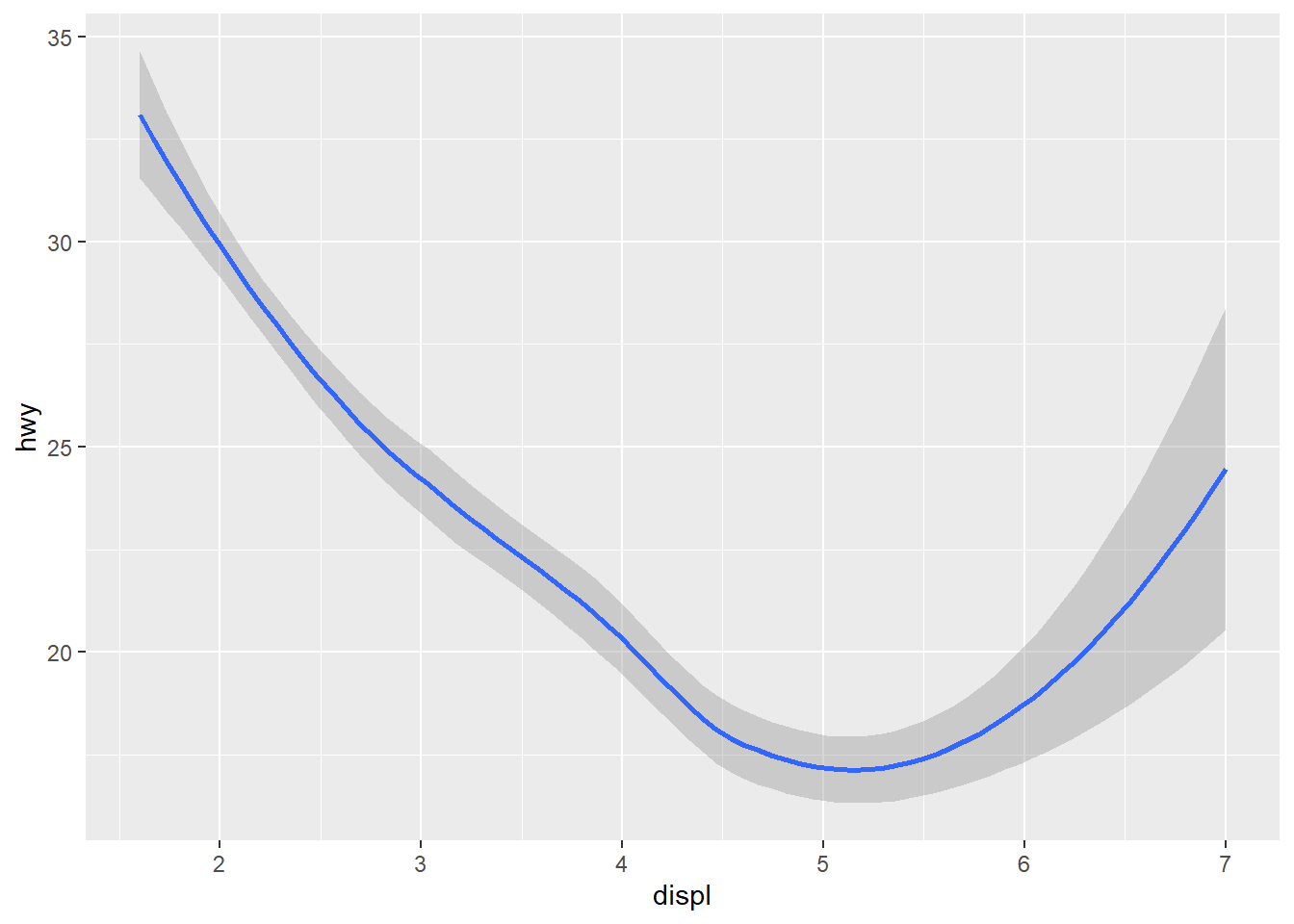ggplot(data = mpg) +   geom_point(mapping = aes(x = displ, y = hwy))ggplot(data = mpg) +   geom_smooth(mapping = aes(x = displ, y = hwy))The above plots are made using the same data, but the geoms are different. To change the plot type i.e. bar, boxplot, line etc. you just change the geom you add to ggplot. The aesthetics that work with each geom is different however. E.g. you may set the linetype of a line, but you can’t change the shape of a line.

ggplot(data = mpg) +  geom_line(aes(x = displ, y = hwy, linetype = drv))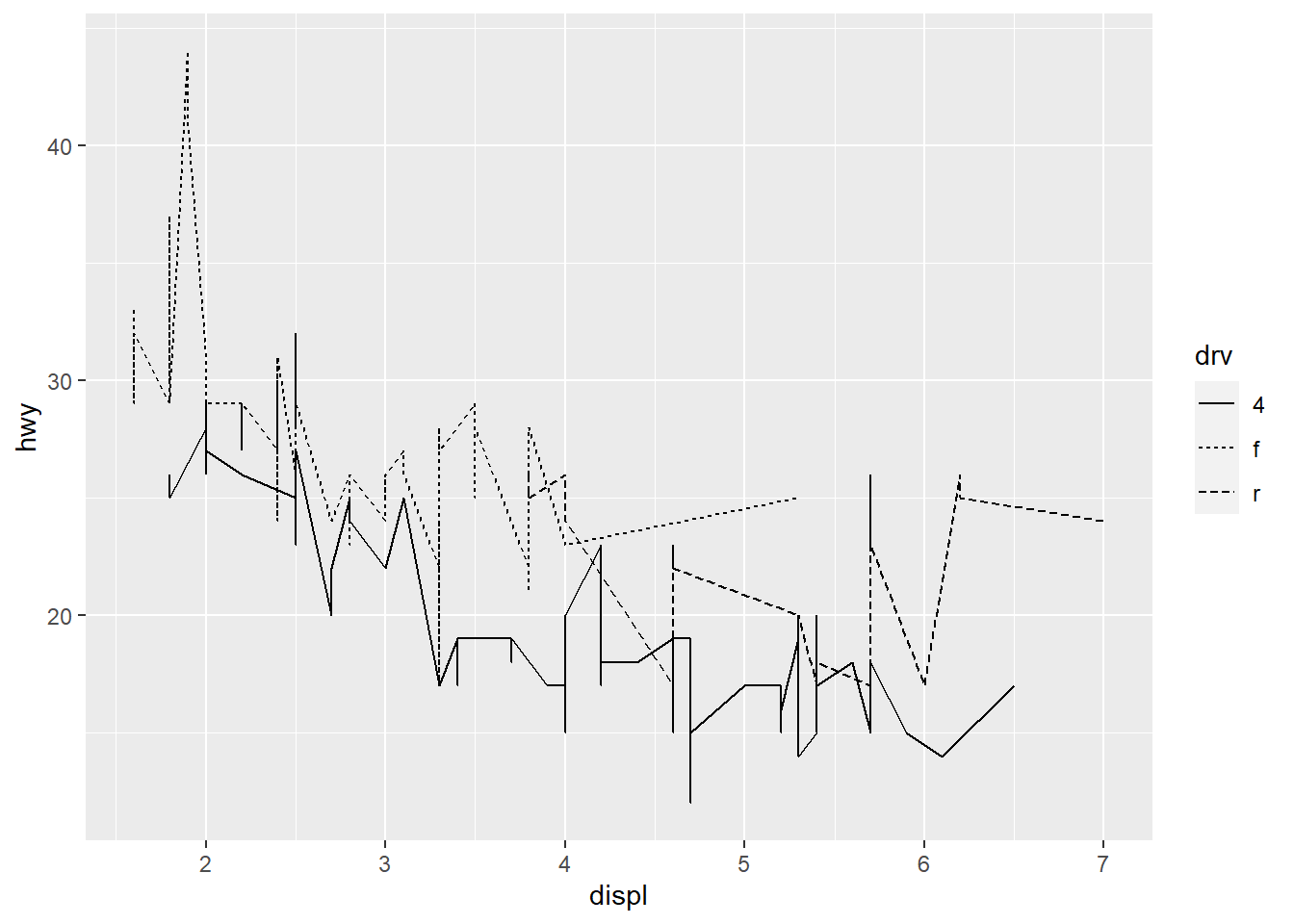ggplot(data = mpg) +  geom_smooth(aes(x = displ, y = hwy, linetype = drv))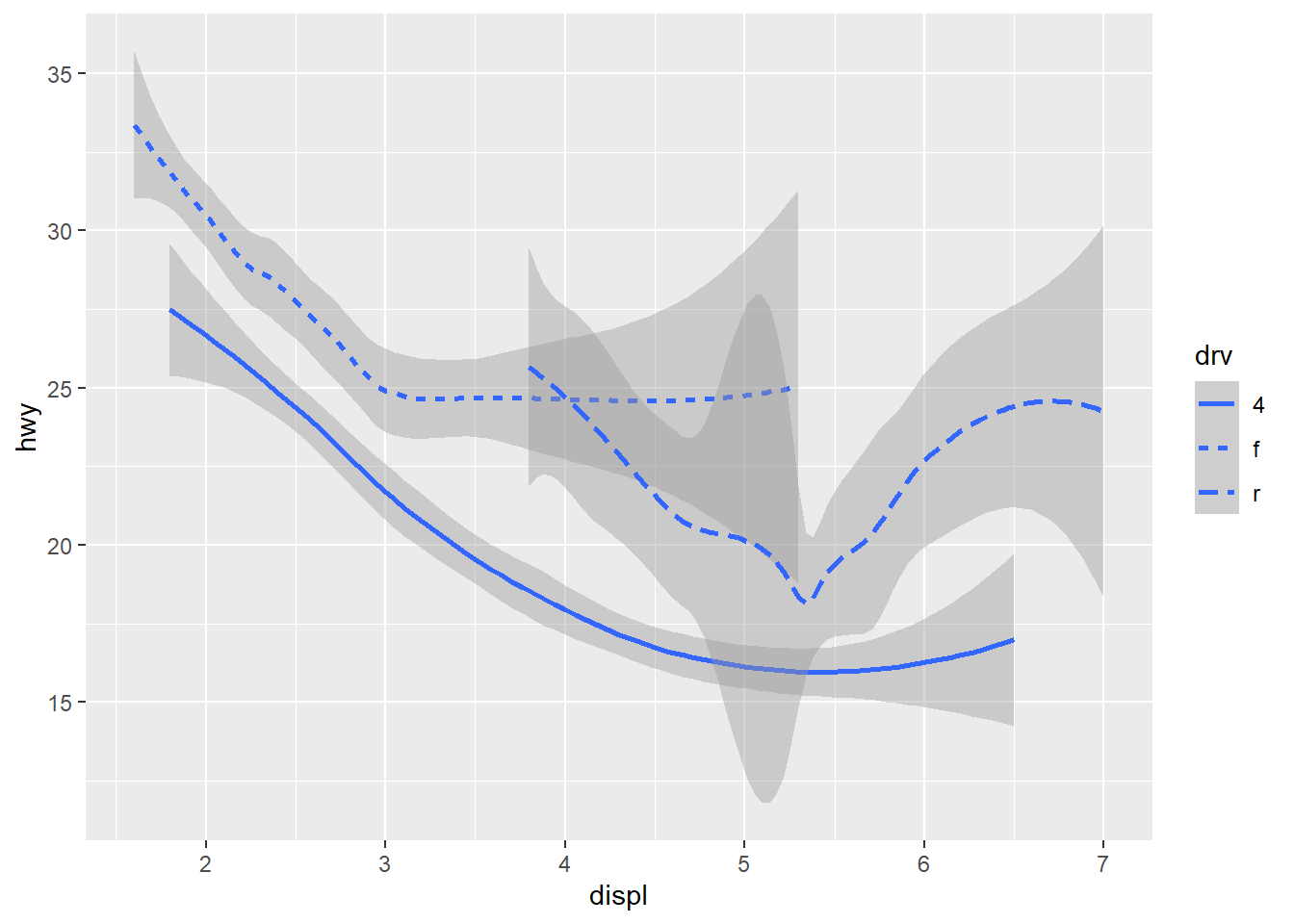ggplot(data = mpg) +  geom_point(mapping = aes(x = displ, y = hwy, colour = drv)) +  geom_smooth(mapping = aes(x = displ, y = hwy, linetype = drv, colour = drv))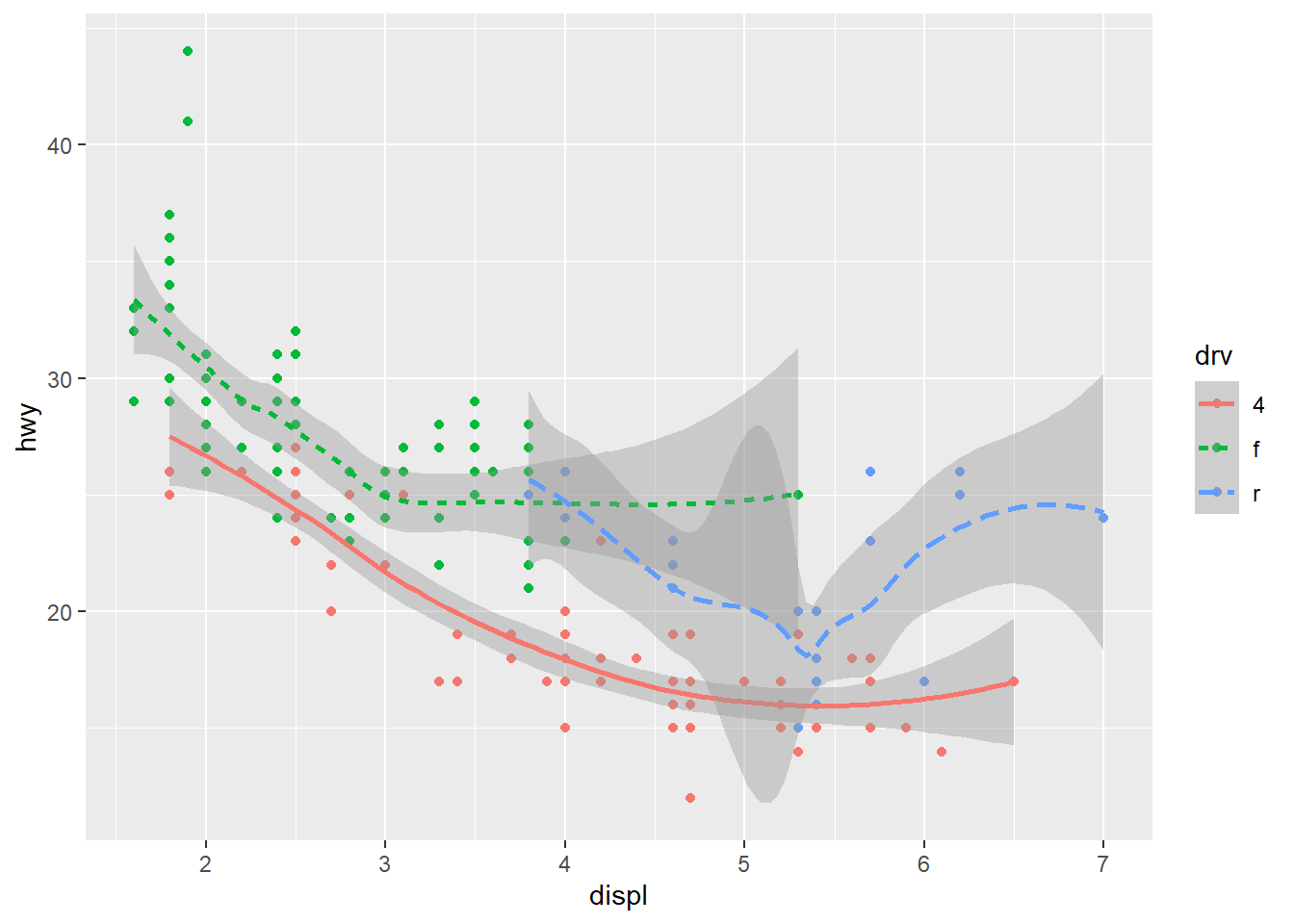We are also able to add more than one geom on a plot. In the above we show both the raw data points coloured by the drive type - e.g. 4 wheel, rear drive or front wheel, as well as each drive types smoothing line. But the downside to the above is that I had to specify the mapping twice with many repeated elements. To avoid this ggplot provides a handy alternate - you may set the mapping in the ggplot() call itself - the mapping is treated as global to all subsequent geoms unless you specifically override these.

ggplot(data = mpg, mapping = aes(x = displ, y = hwy, colour = drv)) +  geom_point() +  geom_smooth(mapping = aes(linetype = drv))Also in the above you may have noticed that geom_smooth() included it’s own mapping. This basically says ‘Hey, take all the attributes as per the ggplot mapping BUT replace these components (if it exists) as described in this geom’.

ggplot(data = mpg, mapping = aes(x = displ, y = hwy)) +  geom_point(mapping = aes(colour = class)) +  geom_smooth()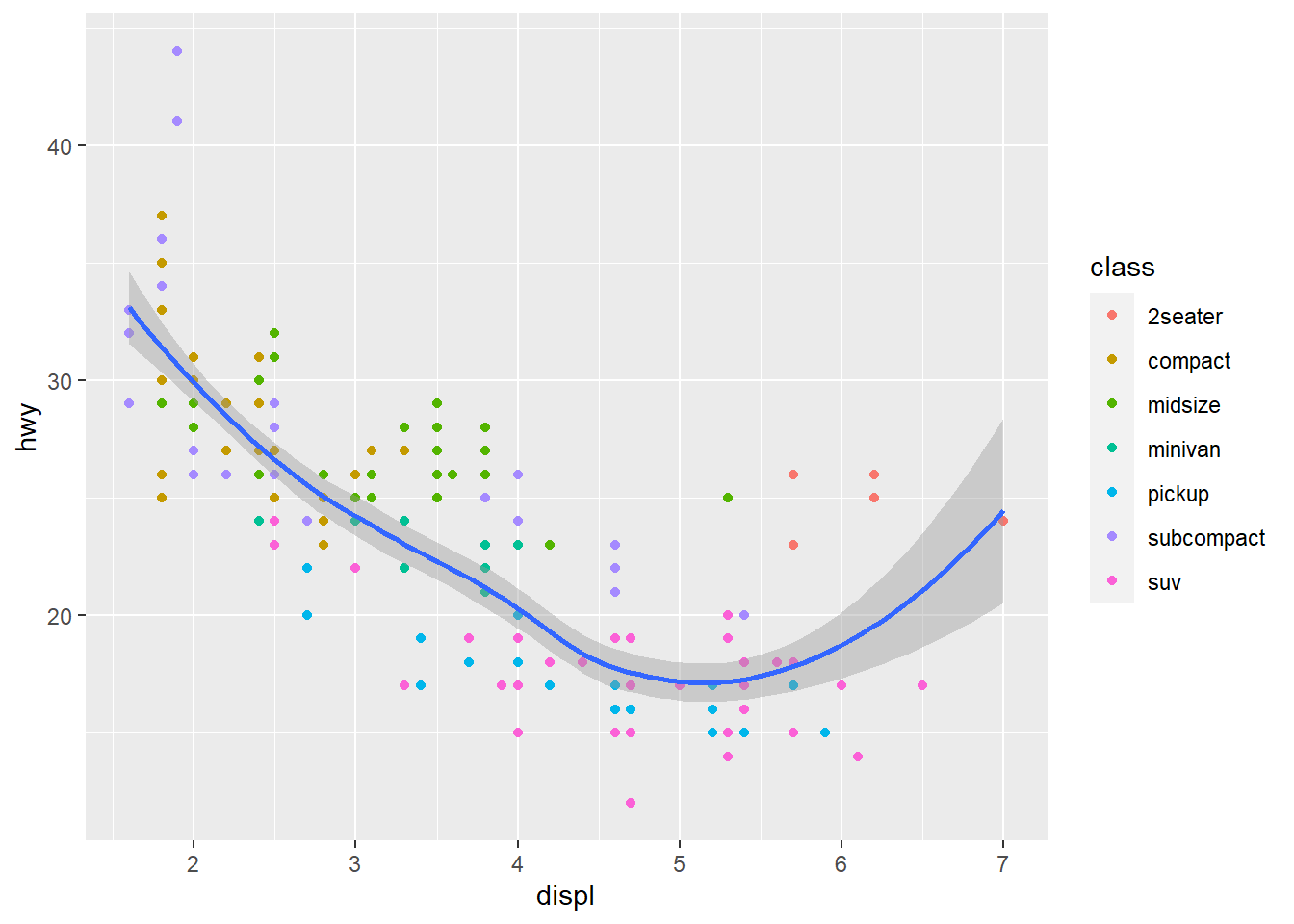For example here when it gets to the geom_point(mapping = aes(colour = class)) line it asks:

1. Does this mapping specify a new x? It doesn’t, so x = displ is set, as per the ggplot() mapping().
2. Does this mapping specify a new y? It doesn’t, so y = hwy is set, as per the ggplot() mapping().
3. Is there anything extra set? Yip, colour = class is set, so let me apply that to this geom_point’s only, hence the scatterpoint plots are coloured by the class of the vehicle.

You may also even change the data for each layer! Here the smoothing function is included but for a subset of the data.

ggplot(data = mpg, aes(x = displ, y = hwy)) +  geom_point(mapping = aes(colour = class)) +  geom_smooth(    data = filter(mpg, class == "subcompact"),    se = FALSE # remove the error bands that display around the line  )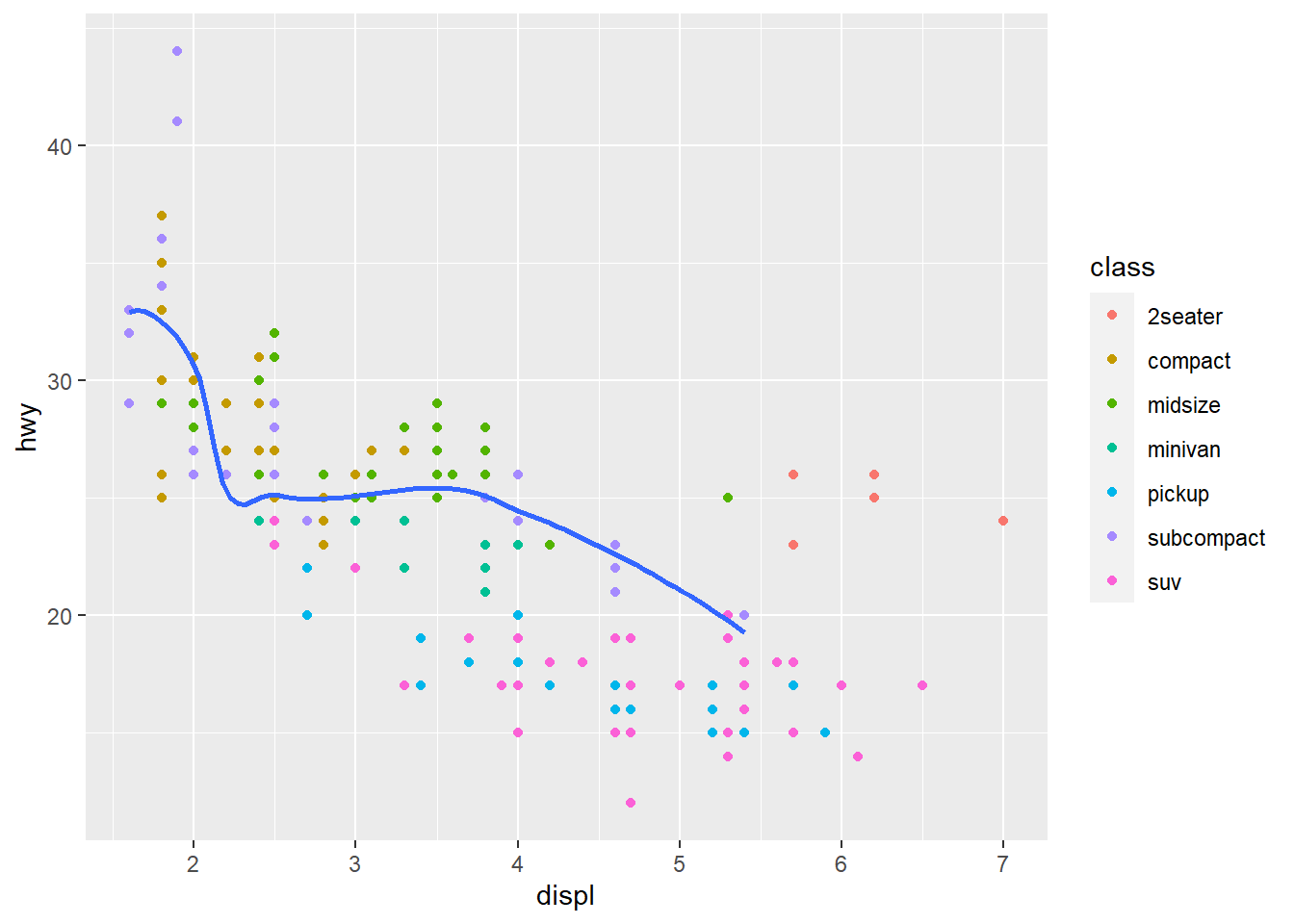### Exercises

1. What geom would you use to draw a line chart? A boxplot? A histogram? An area chart?

• line chart: geom_line()
• boxplot: geom_boxplot()
• histogram: geom_histogram()
• Area chart: geom_area()
2. Run this code in your head and predict what the output will look like. Then, run the code in R and check your predictions.

ggplot(data = mpg, mapping = aes(x = displ, y = hwy, color = drv)) +
geom_point() +
geom_smooth(se = FALSE)

In my head this will produce a scatterplot with displ on the x-axis, hwy on the y-axis where each point is coloured by the type of drive the vehicle is (4 wheel, front-wheel, rear-wheel). The plot will also contain smoothing lines (with no error bands) for each drive type (since colour is set in the ggplot mapping layer, and is applicable for both points, and the smoothing lines).

ggplot(data = mpg, mapping = aes(x = displ, y = hwy, color = drv)) +
geom_point() +
geom_smooth(se = FALSE)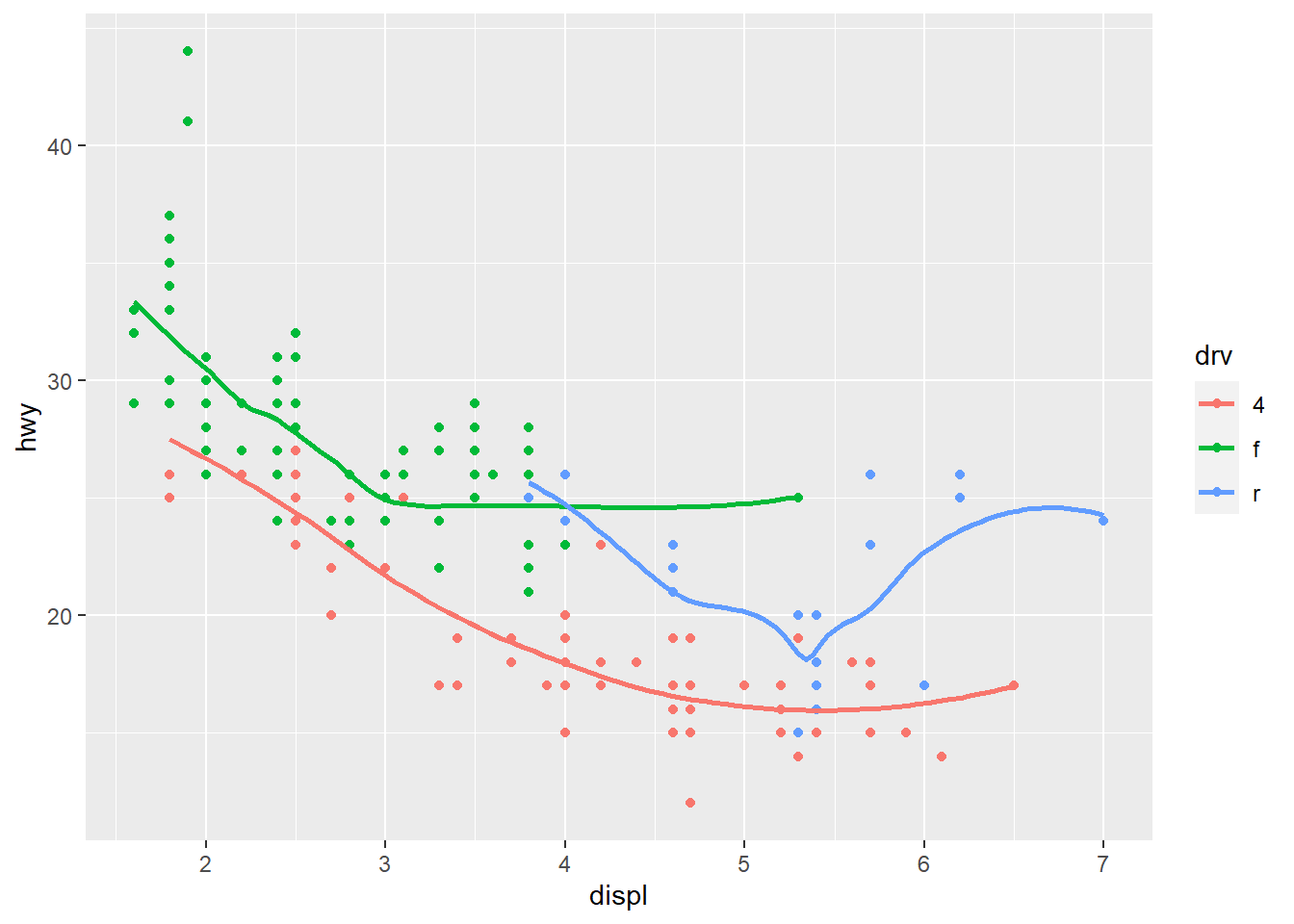3. What does show.legend = FALSE do? What happens if you remove it?
Why do you think I used it earlier in the chapter?

It removes the legend that shows up when we add certain aesthetics such as colour, shape etc.

If we remove it the legend will show by the fact that a certain non-xy aesthetic has been added.

It was used to remove the legend earlier which would have shown up due to the colour = drv aesthetic.

4. What does the se argument to geom_smooth() do? It sets the errors bands on the smoothing function to either on or off.

5. Will these two graphs look different? Why/why not?

ggplot(data = mpg, mapping = aes(x = displ, y = hwy)) +
geom_point() +
geom_smooth()

ggplot() +
geom_point(data = mpg, mapping = aes(x = displ, y = hwy)) +
geom_smooth(data = mpg, mapping = aes(x = displ, y = hwy))

They will look the same. In the first plot the data = mpg, mapping = aes(x = displ, y = hwy) will be inherited by both geom_point() and geom_smooth(). In the second plot the data = mpg, mapping = aes(x = displ, y = hwy) is repeated in each geom and hence both plots will be the same.

ggplot(data = mpg, mapping = aes(x = displ, y = hwy)) +
geom_point() +
geom_smooth()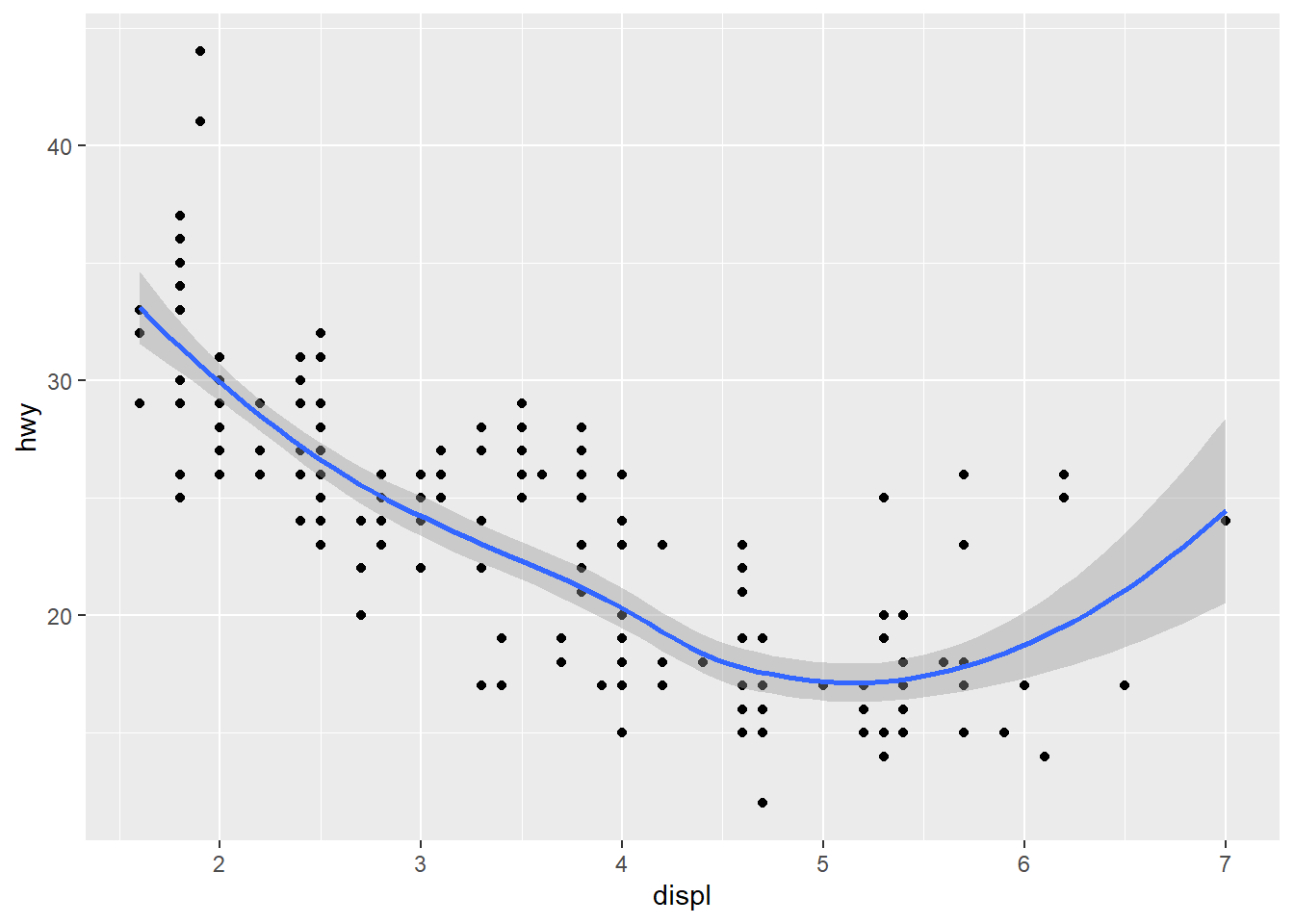ggplot() +
geom_point(data = mpg, mapping = aes(x = displ, y = hwy)) +
geom_smooth(data = mpg, mapping = aes(x = displ, y = hwy))6. Recreate the R code necessary to generate the following graphs.

ggplot(data = mpg, aes(x = displ, y = hwy)) +
geom_point(size = 2) +
geom_smooth(se = FALSE)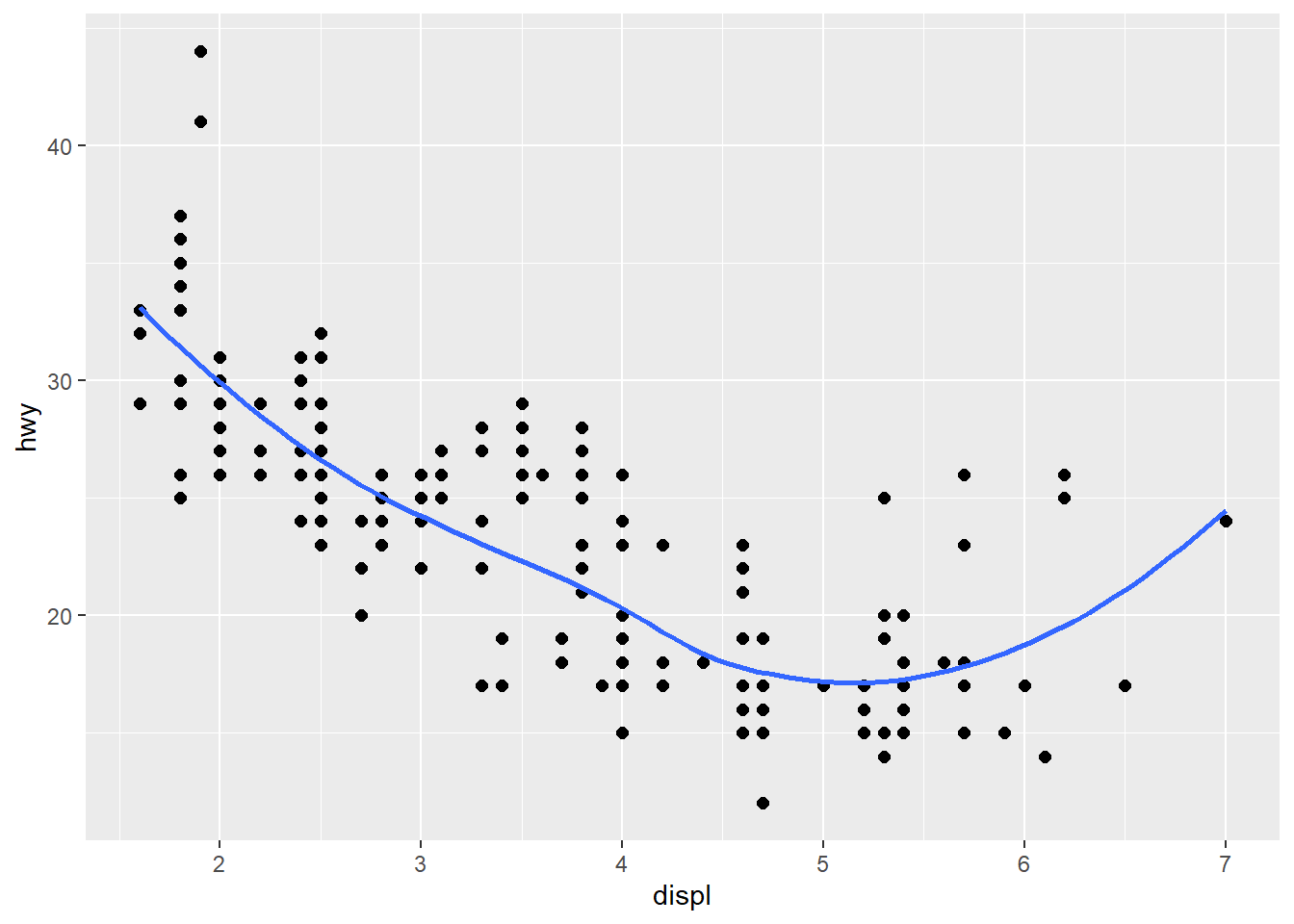ggplot(data = mpg, aes(x = displ, y = hwy)) +
geom_point(size = 2) +
geom_smooth(aes(group = drv), se = FALSE)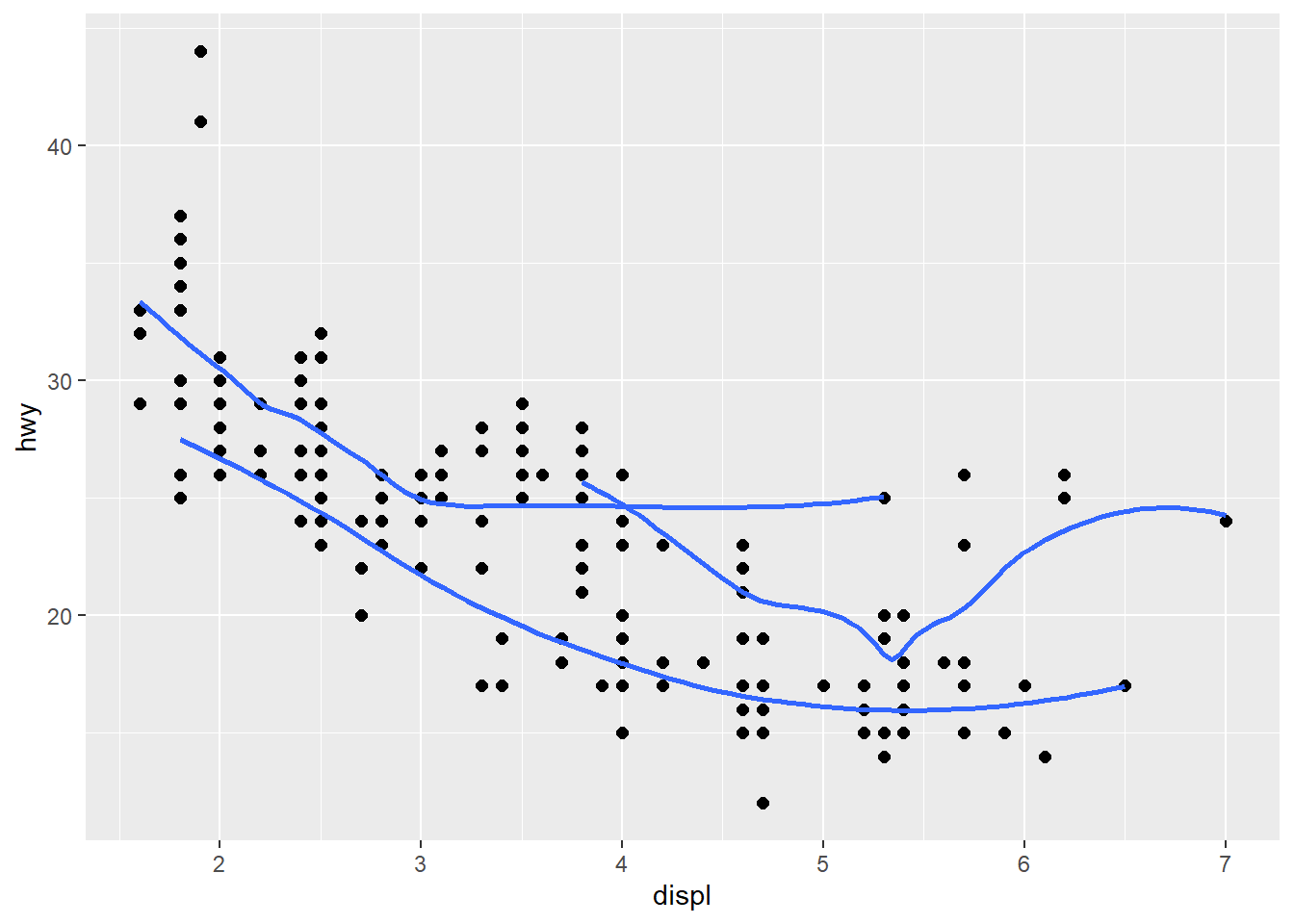ggplot(data = mpg, aes(x = displ, y = hwy, colour = drv)) +
geom_point(size = 2) +
geom_smooth(se = FALSE)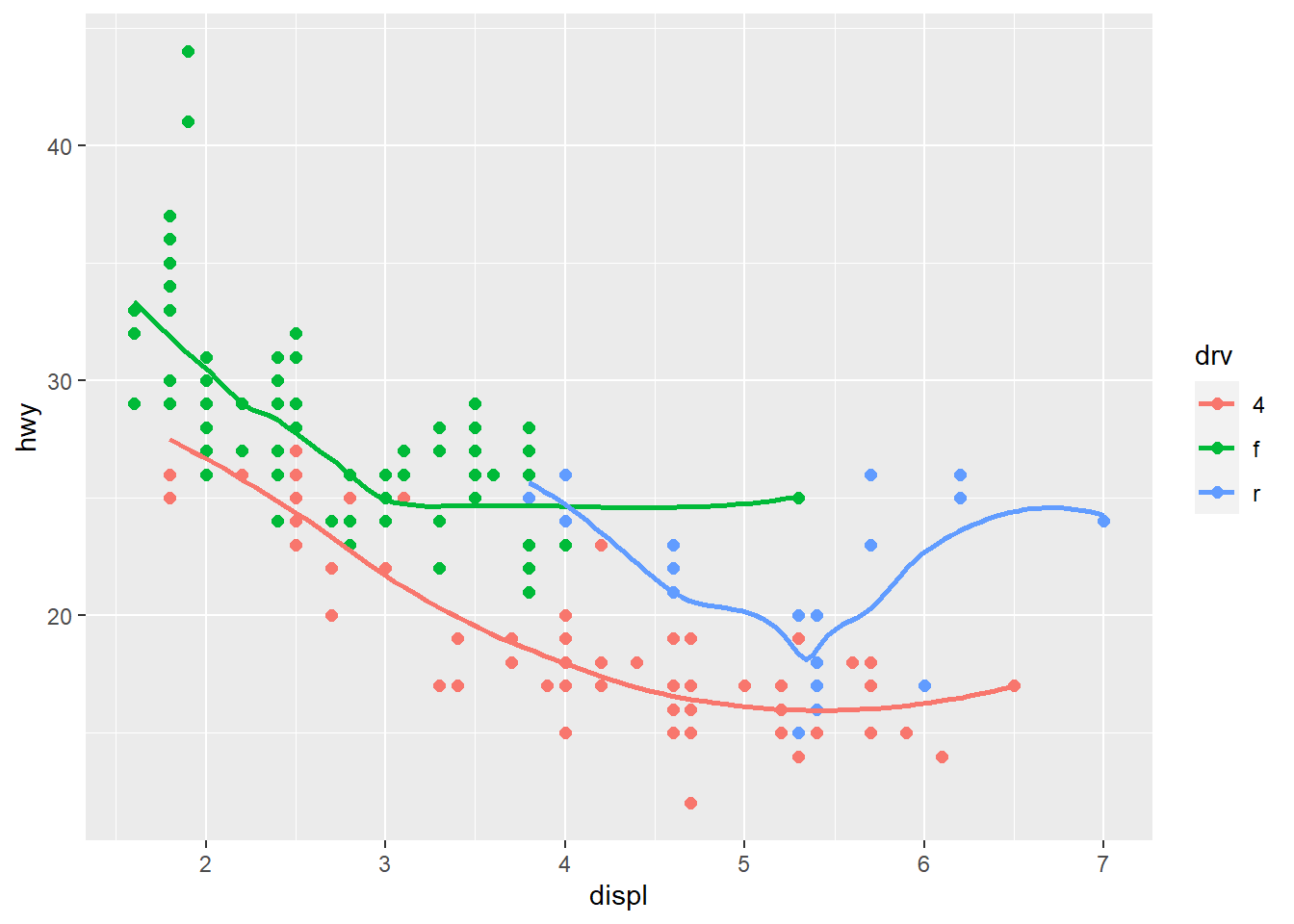ggplot(data = mpg, aes(x = displ, y = hwy)) +
geom_point(aes(colour = drv)) +
geom_smooth(se = FALSE)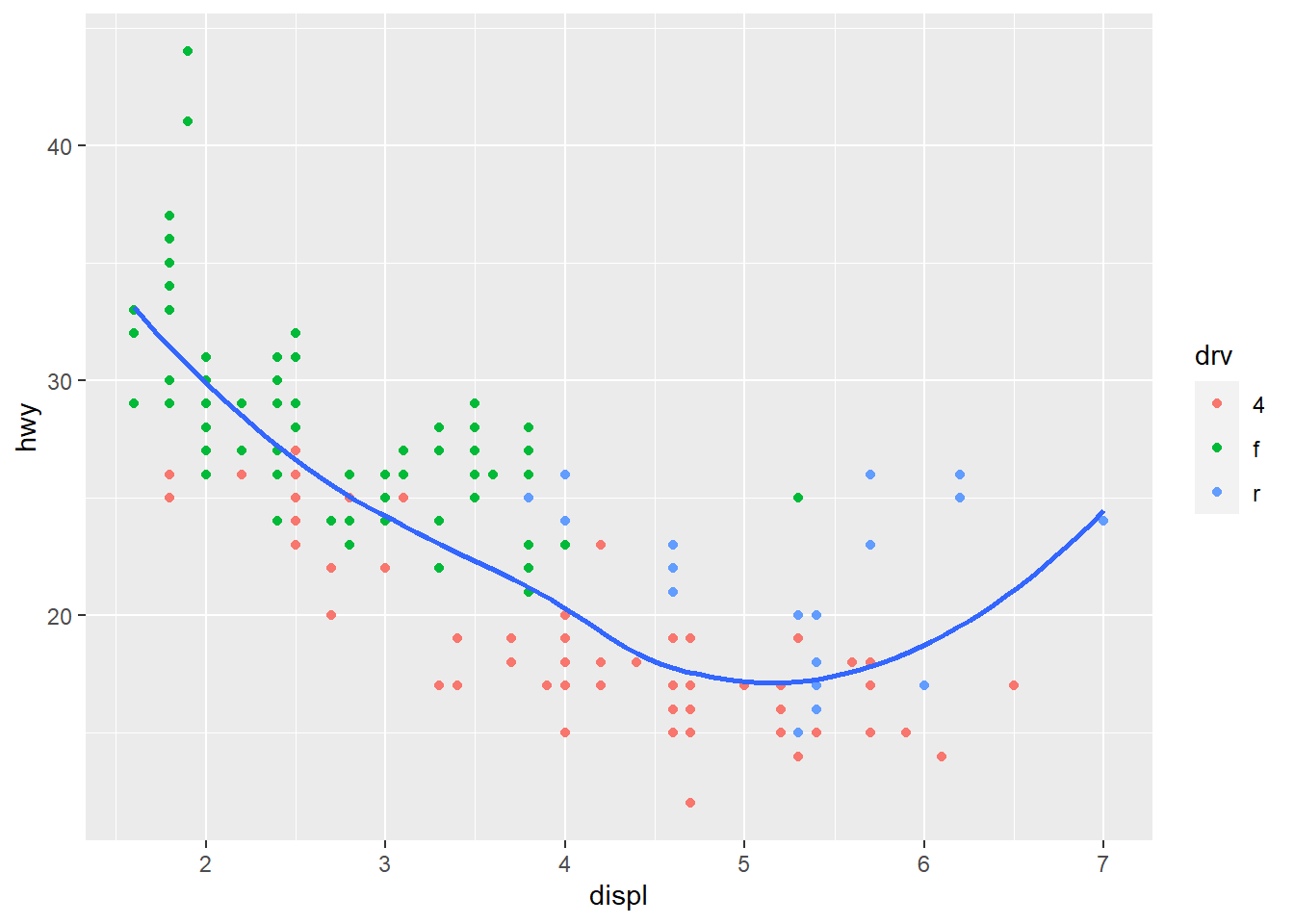ggplot(data = mpg, aes(x = displ, y = hwy)) +
geom_point(aes(colour = drv)) +
geom_smooth(aes(linetype = drv), se = FALSE)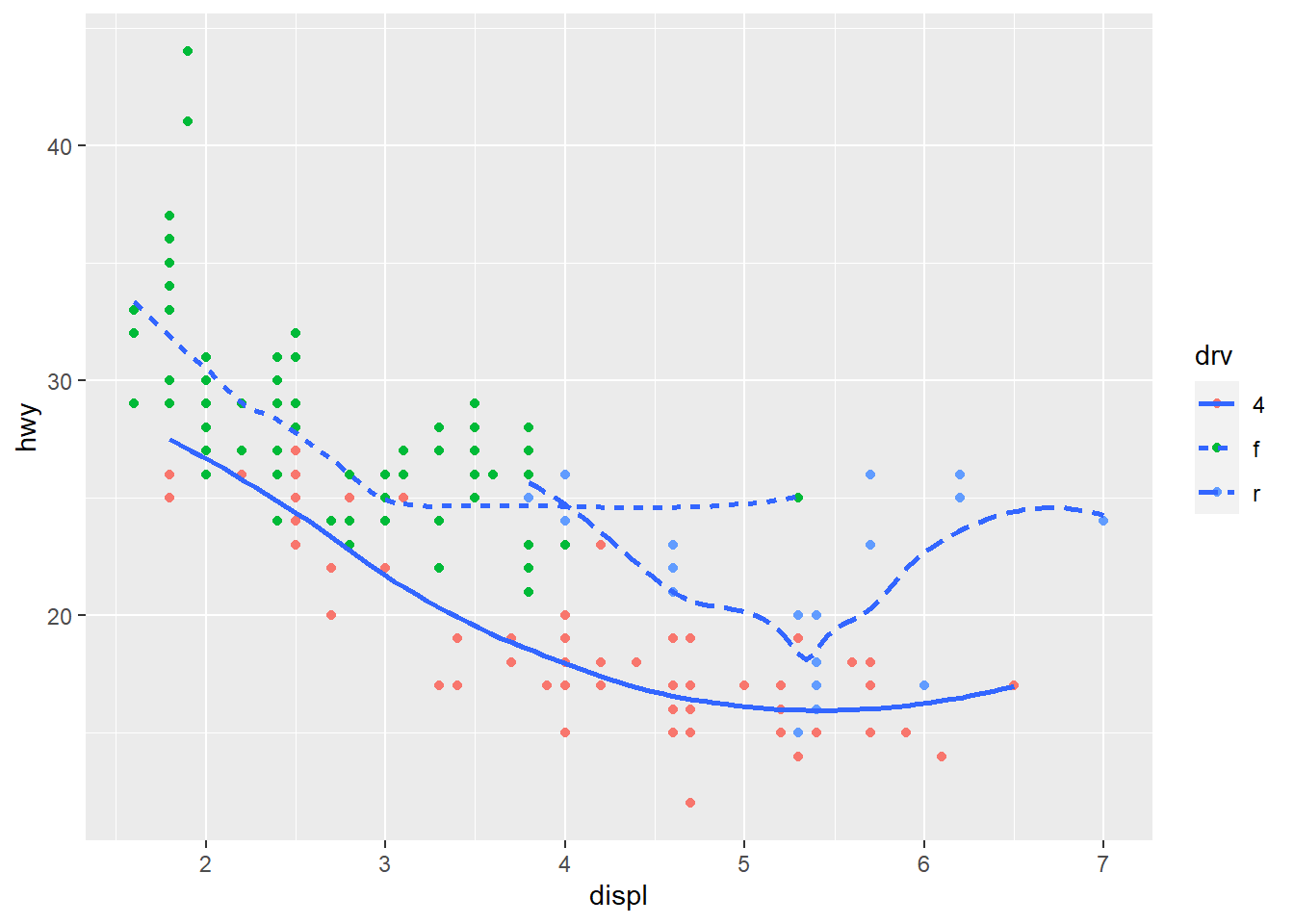ggplot(data = mpg, aes(x = displ, y = hwy)) +
geom_point(size = 3, colour = "white") +
geom_point(aes(colour = drv))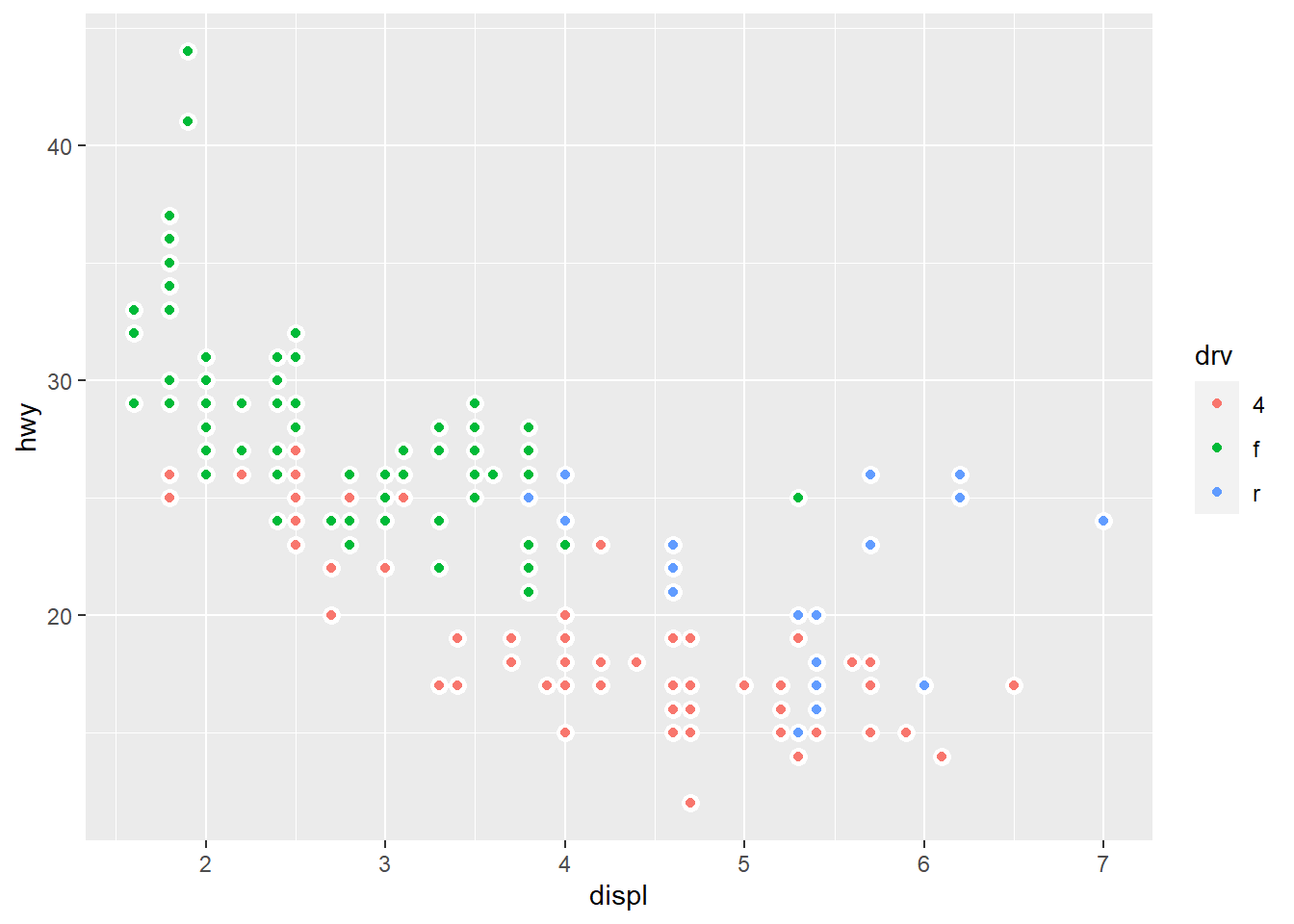sessionInfo()
R version 3.6.3 (2020-02-29)
Platform: x86_64-w64-mingw32/x64 (64-bit)
Running under: Windows 10 x64 (build 18363)

Matrix products: default

locale:
 LC_COLLATE=English_South Africa.1252  LC_CTYPE=English_South Africa.1252
 LC_MONETARY=English_South Africa.1252 LC_NUMERIC=C
 LC_TIME=English_South Africa.1252

attached base packages:
 stats     graphics  grDevices utils     datasets  methods   base

other attached packages:
 flair_0.0.2     forcats_0.5.0   stringr_1.4.0   dplyr_1.0.0
 ggplot2_3.3.0   tidyverse_1.3.0 workflowr_1.6.2

loaded via a namespace (and not attached):
 Rcpp_1.0.4.6     lubridate_1.7.8  lattice_0.20-38  assertthat_0.2.1
 rprojroot_1.3-2  digest_0.6.25    utf8_1.1.4       R6_2.4.1
 cellranger_1.1.0 backports_1.1.6  reprex_0.3.0     evaluate_0.14
 rvest_0.3.5      knitr_1.28       haven_2.2.0